# RD Sharma Solutions for Class 6 Maths Chapter 7: Decimals

RD Sharma Solutions is a valuable resource which can be used by students to perform well in the exam. Decimals is one of the important concepts in Mathematics and the students need to pay much attention in understanding them. The primary mission of providing chapter wise detailed solutions is to make the students tension free before appearing for exams.

In order to reduce the fear of forgetting the methods used in solving problems, our expert faculty create solutions with explanations in a simple language. The students can download the PDF of solutions either chapter wise or exercise wise, based on the requirement. RD Sharma Solutions for Class 6 Maths Chapter 7 Decimals are provided here.

## RD Sharma Solutions for Class 6 Maths Chapter 7: Decimals Download PDF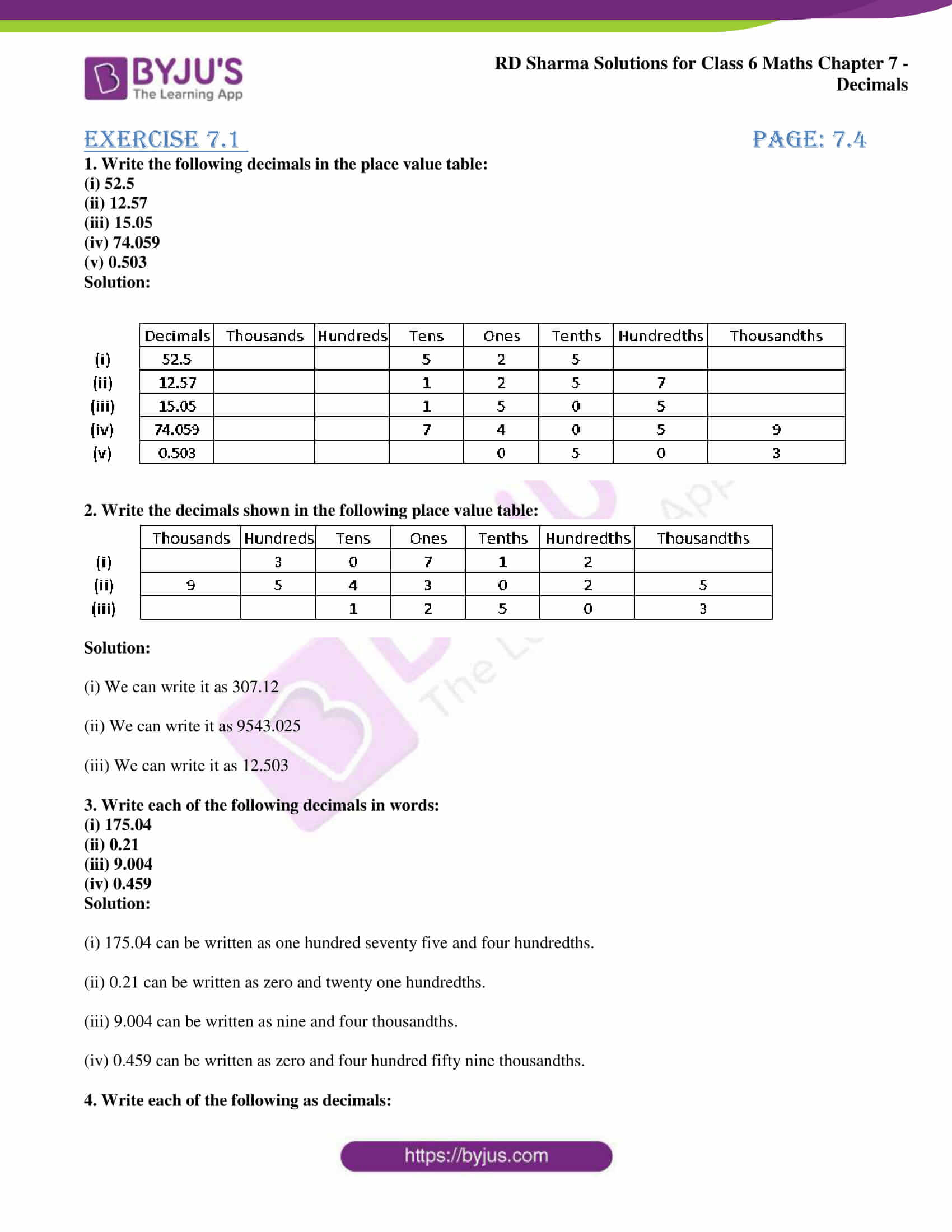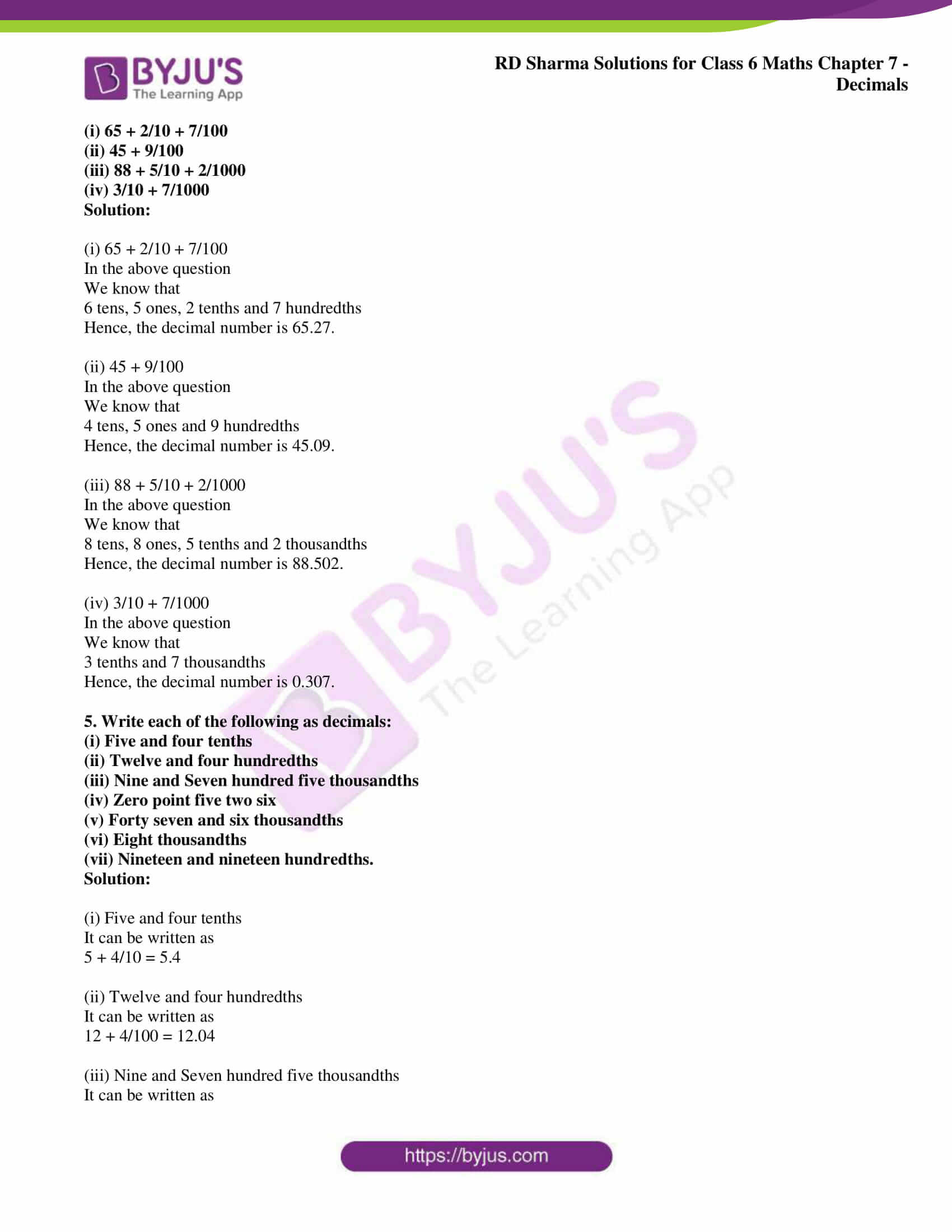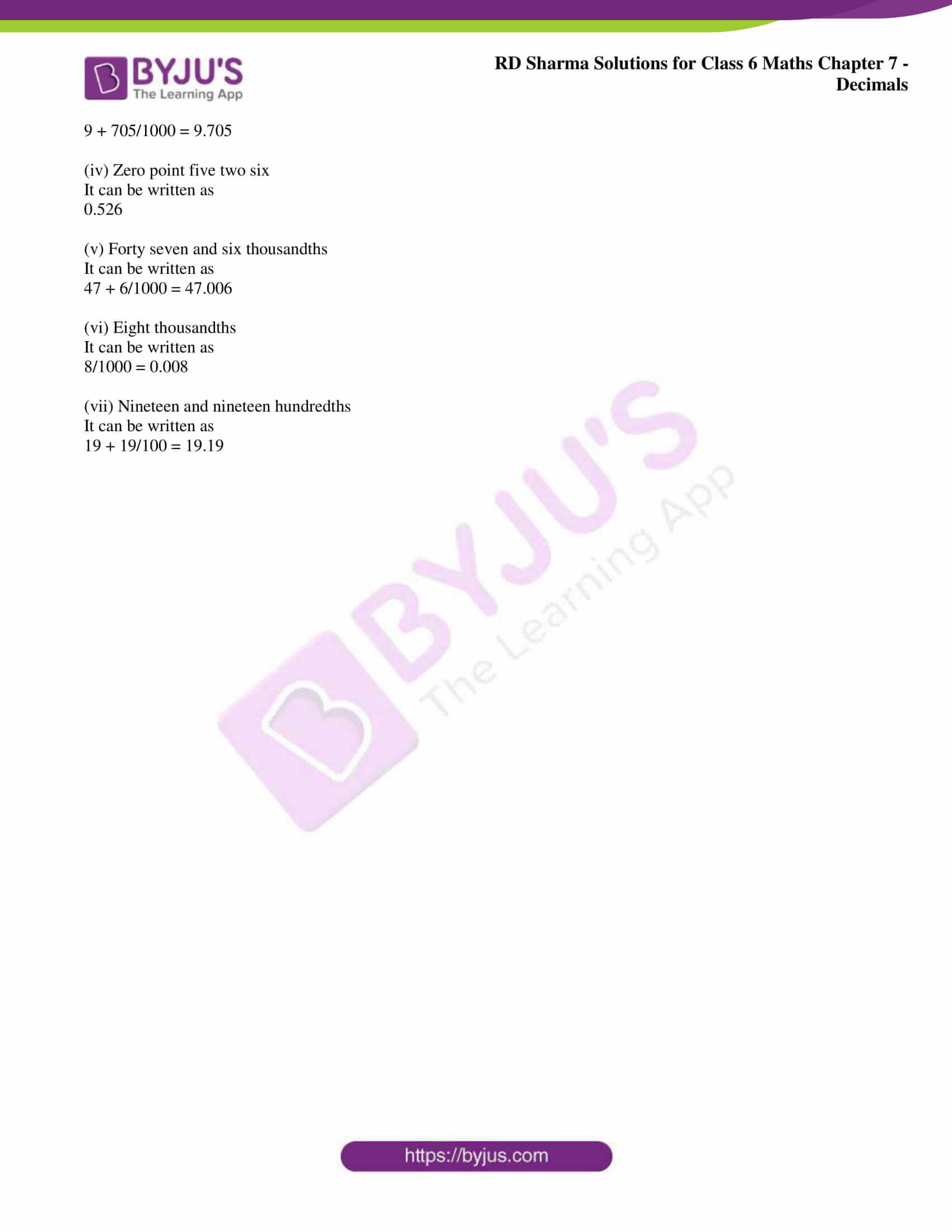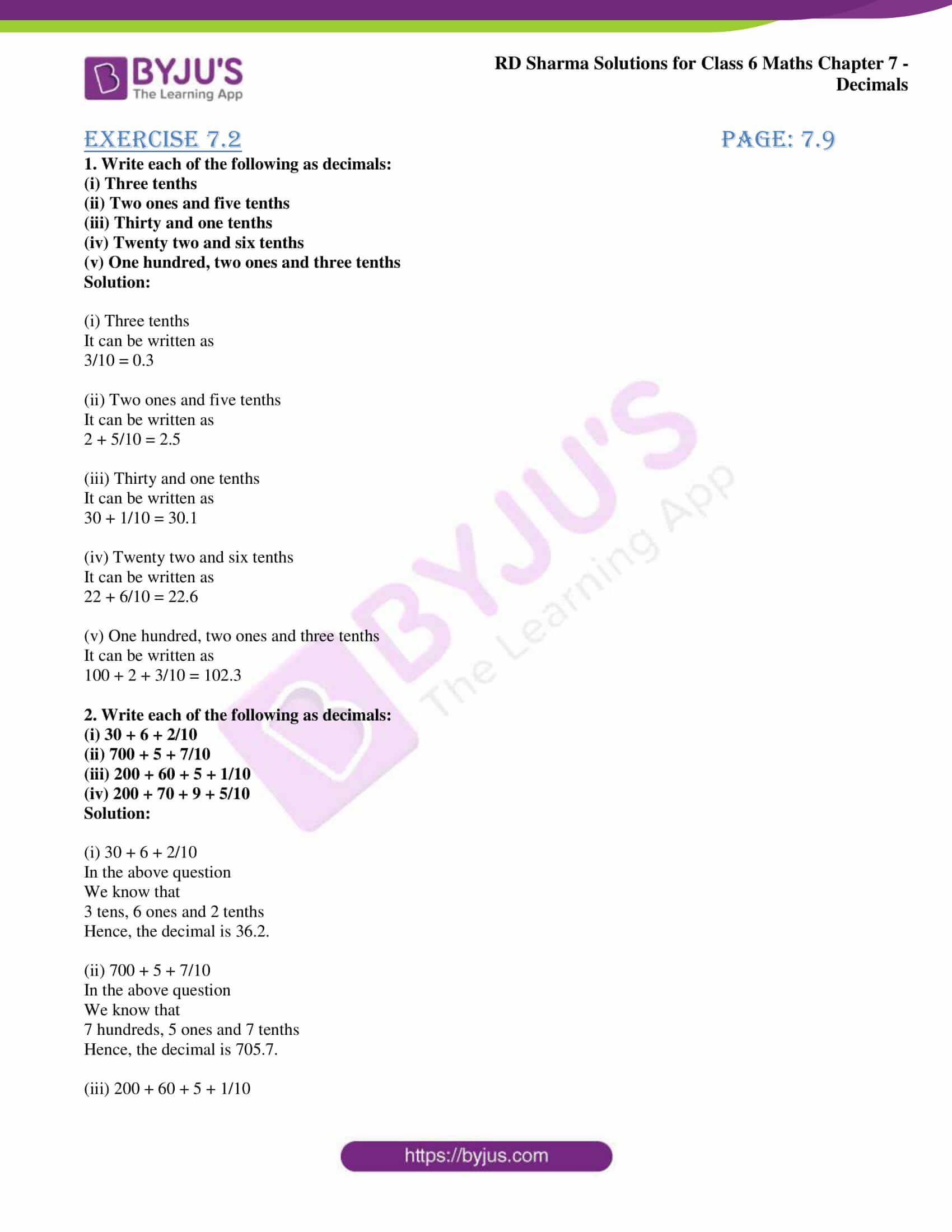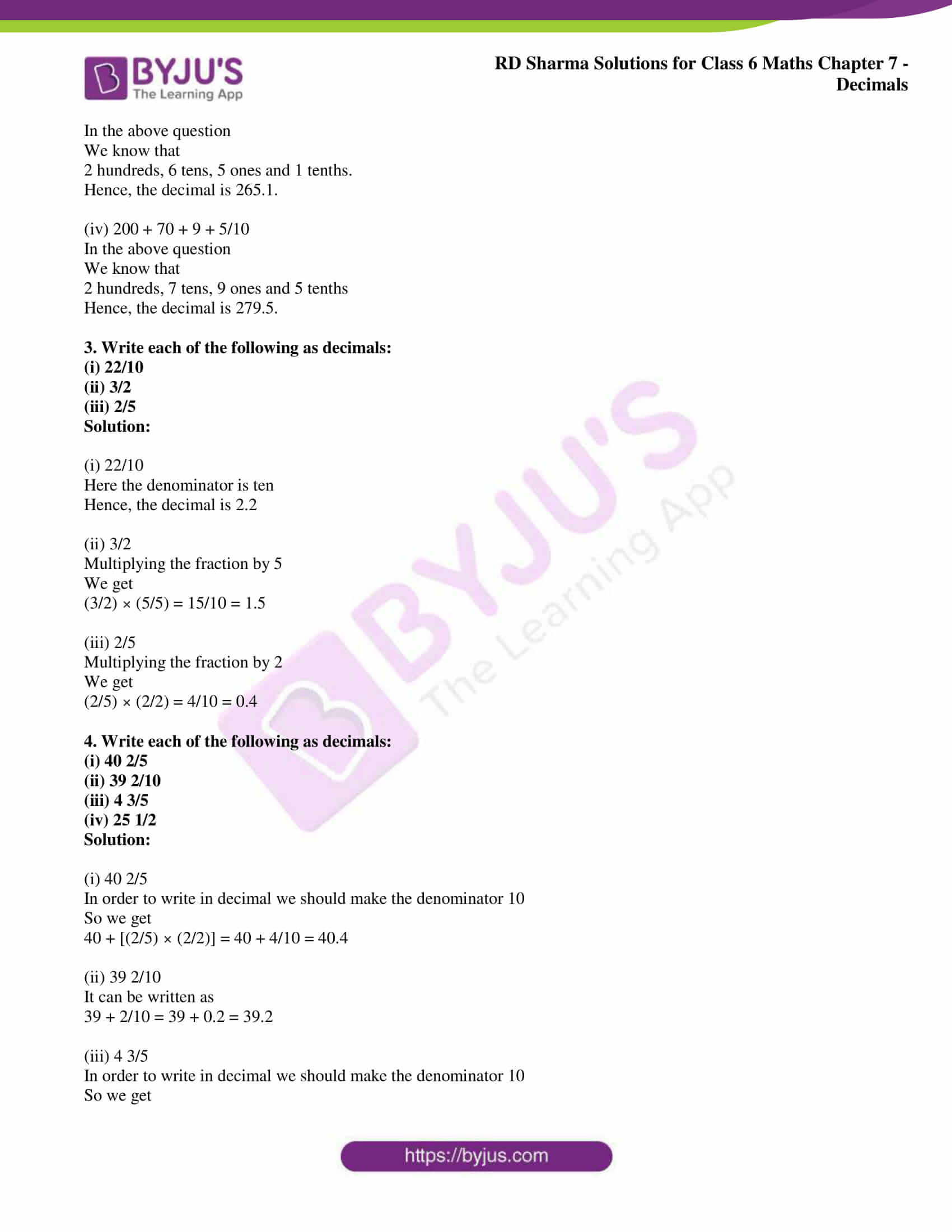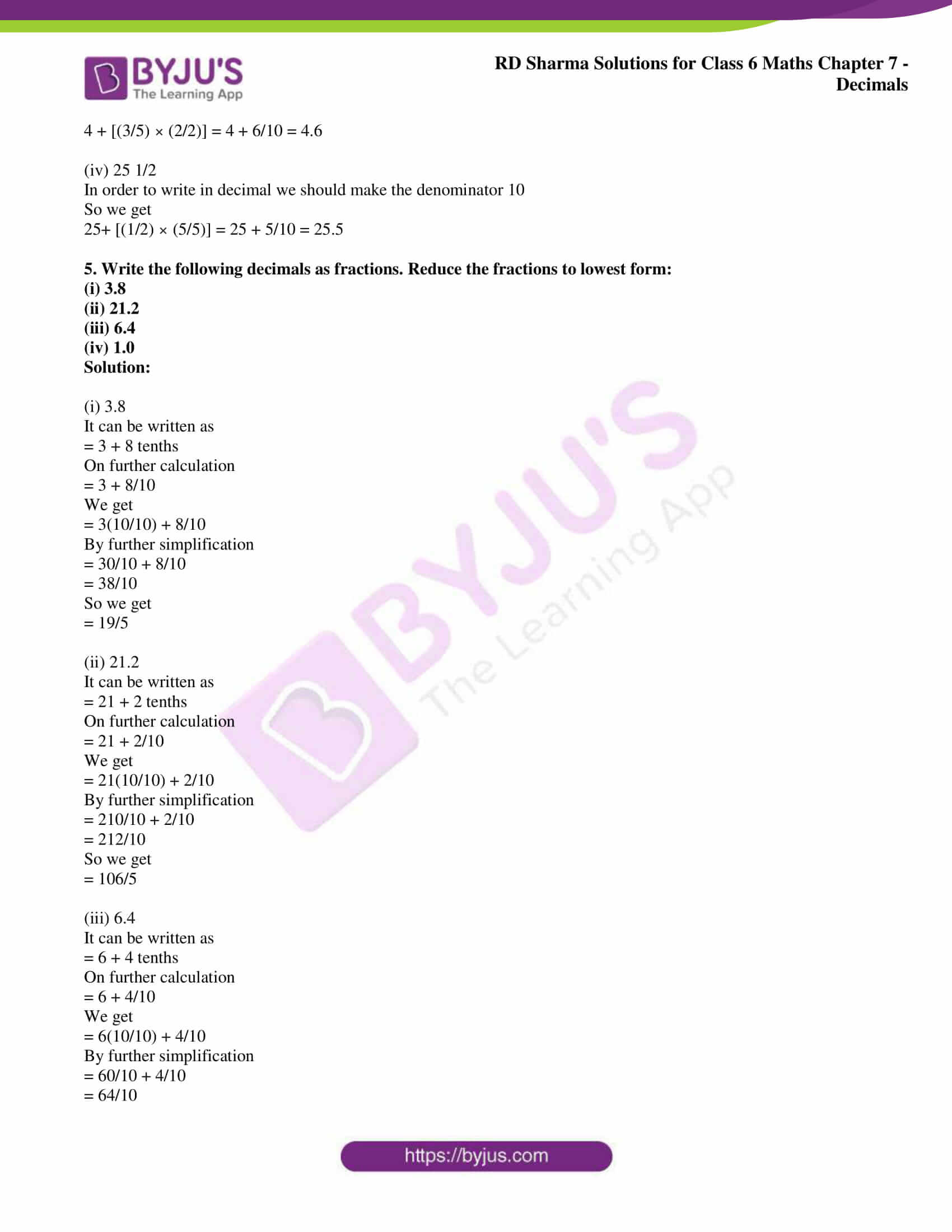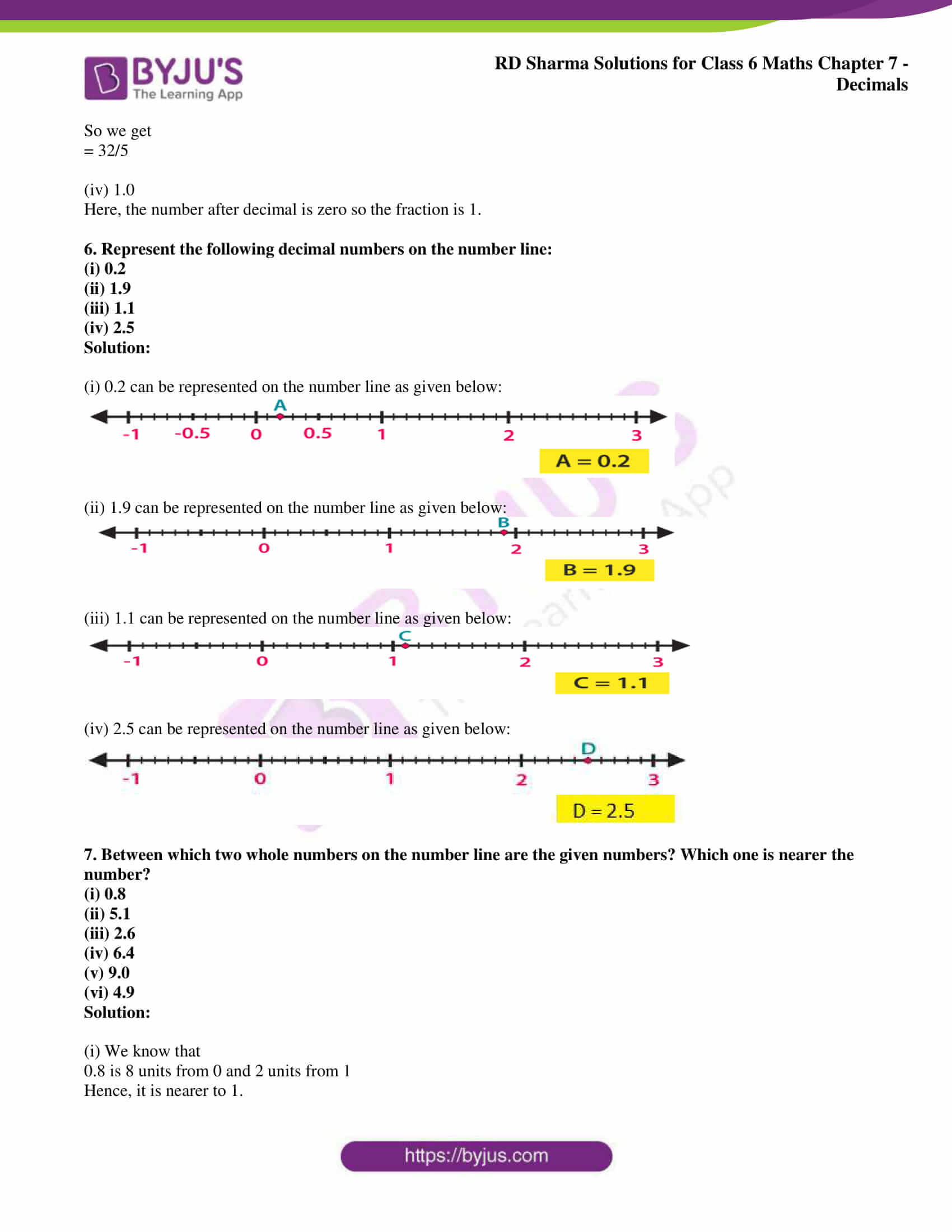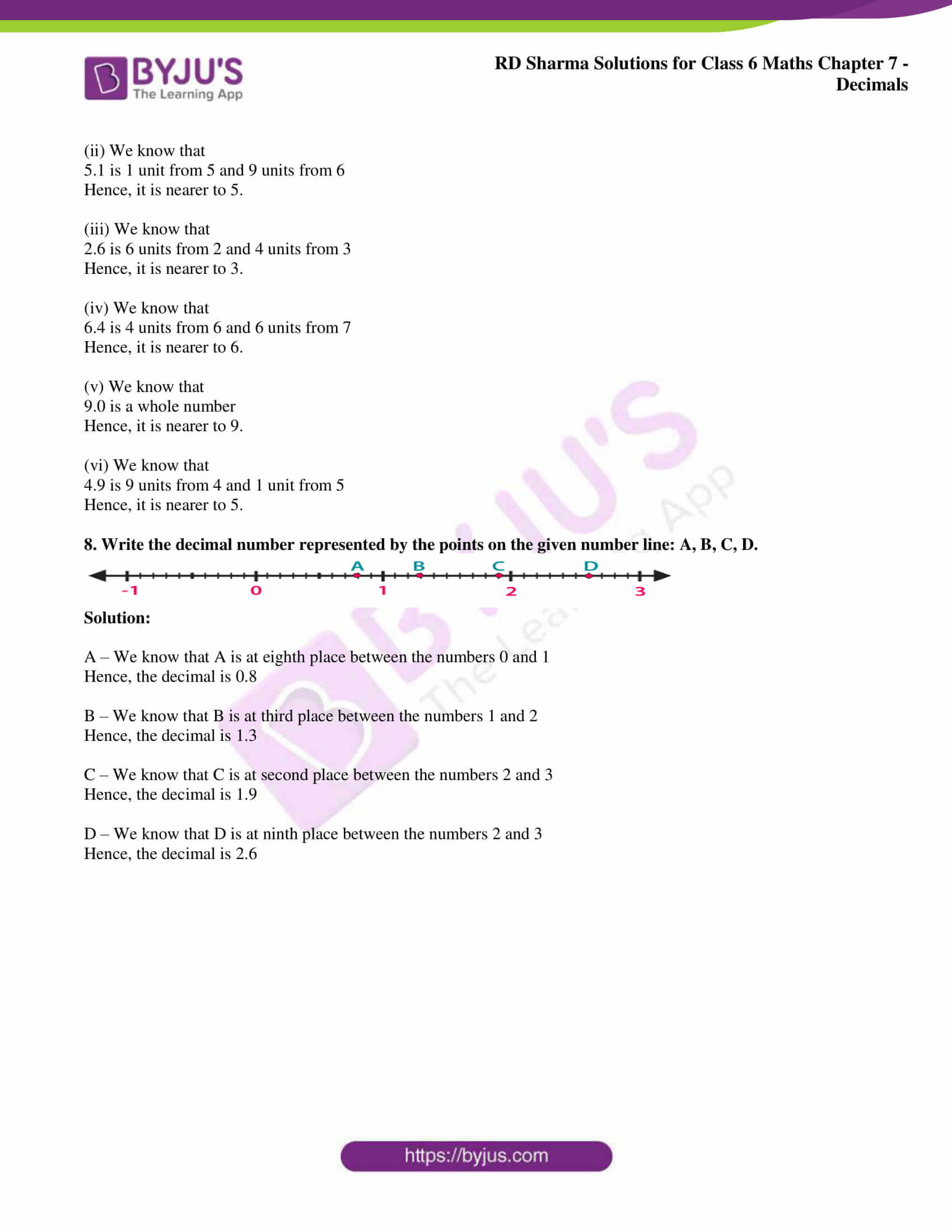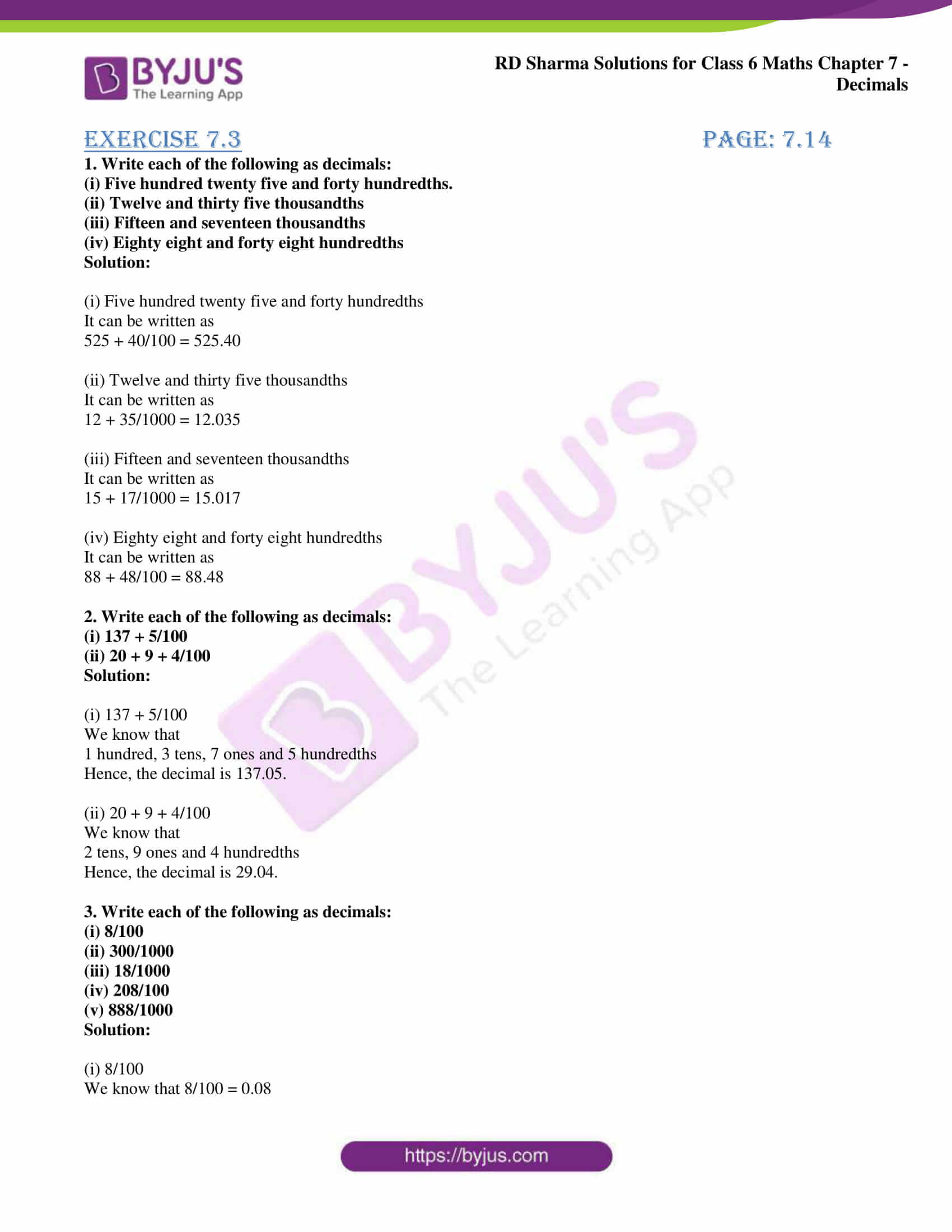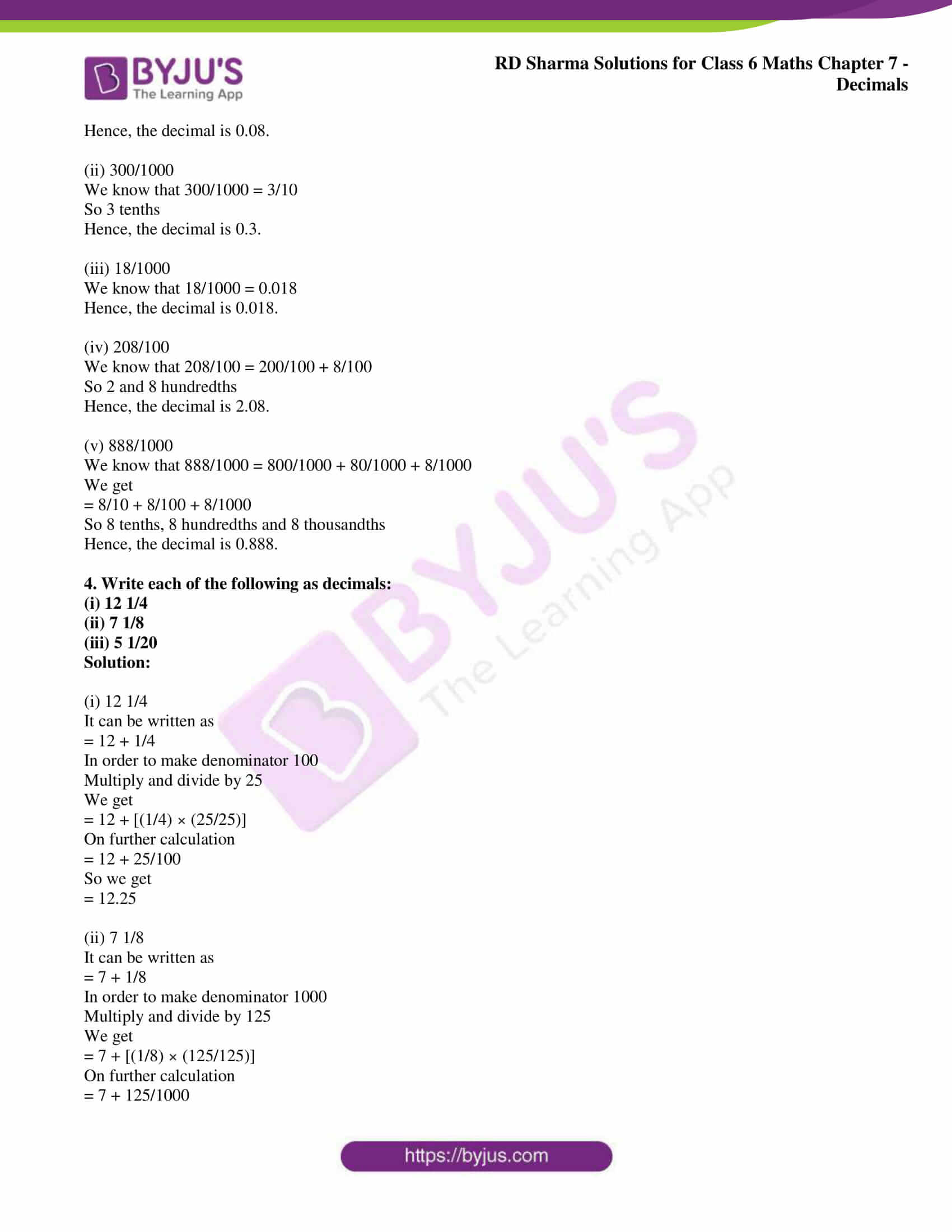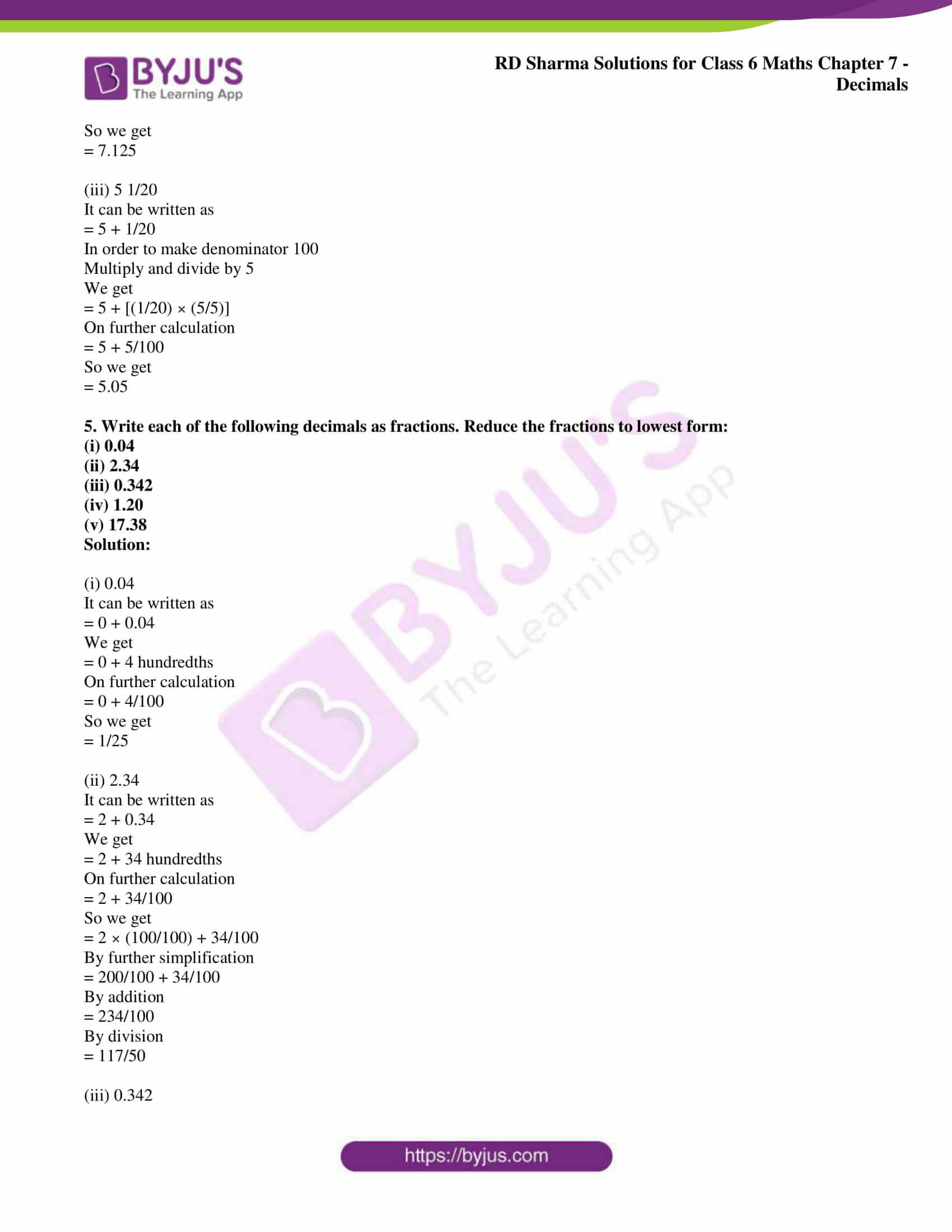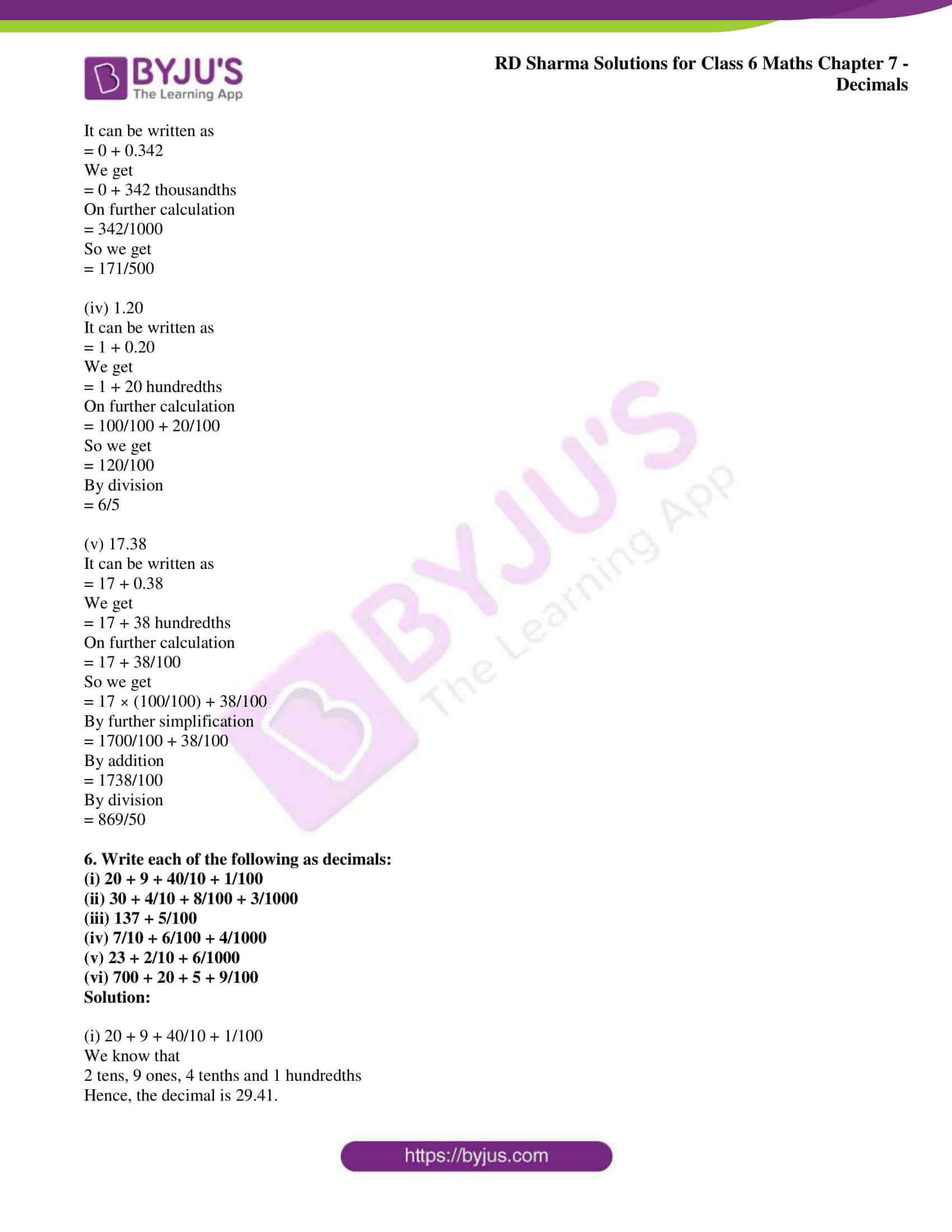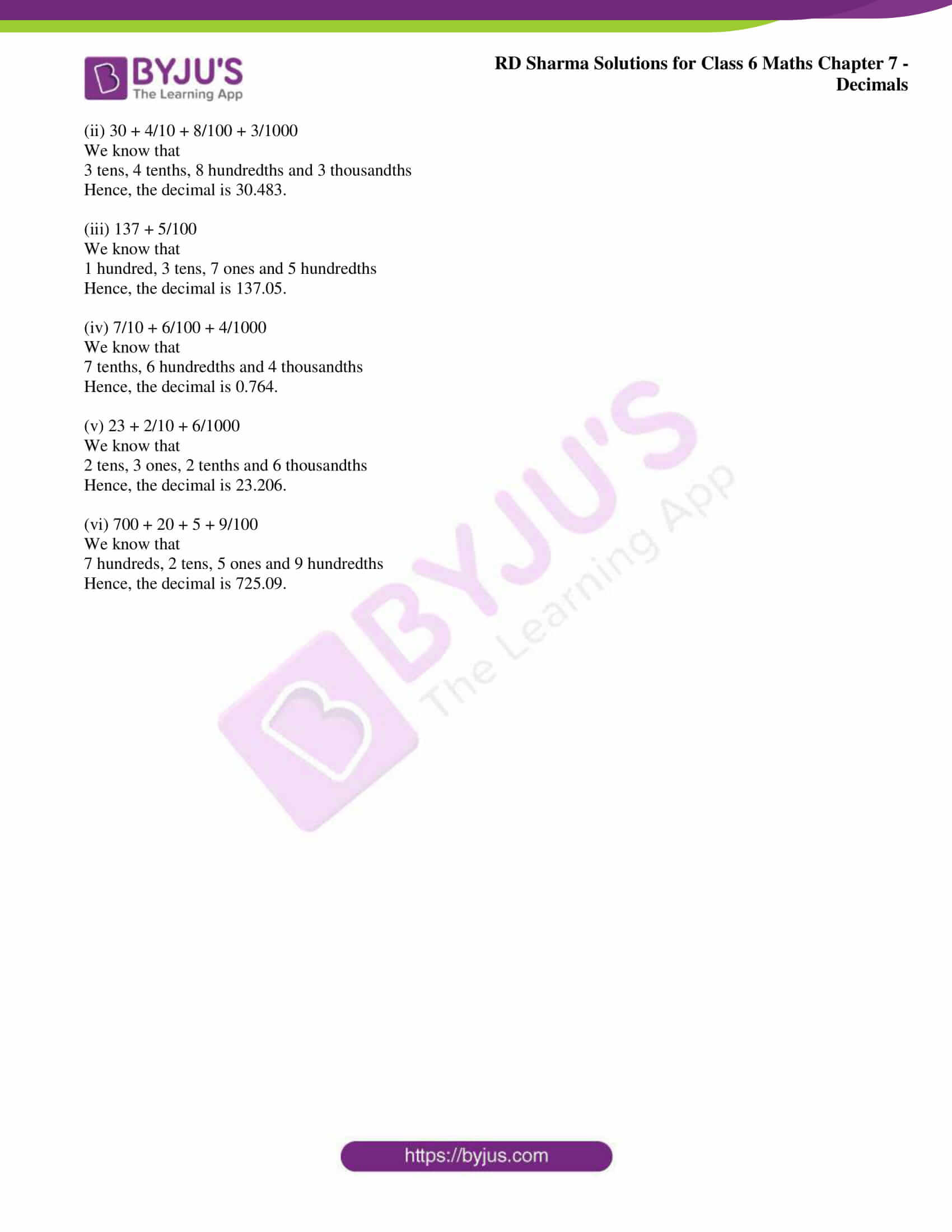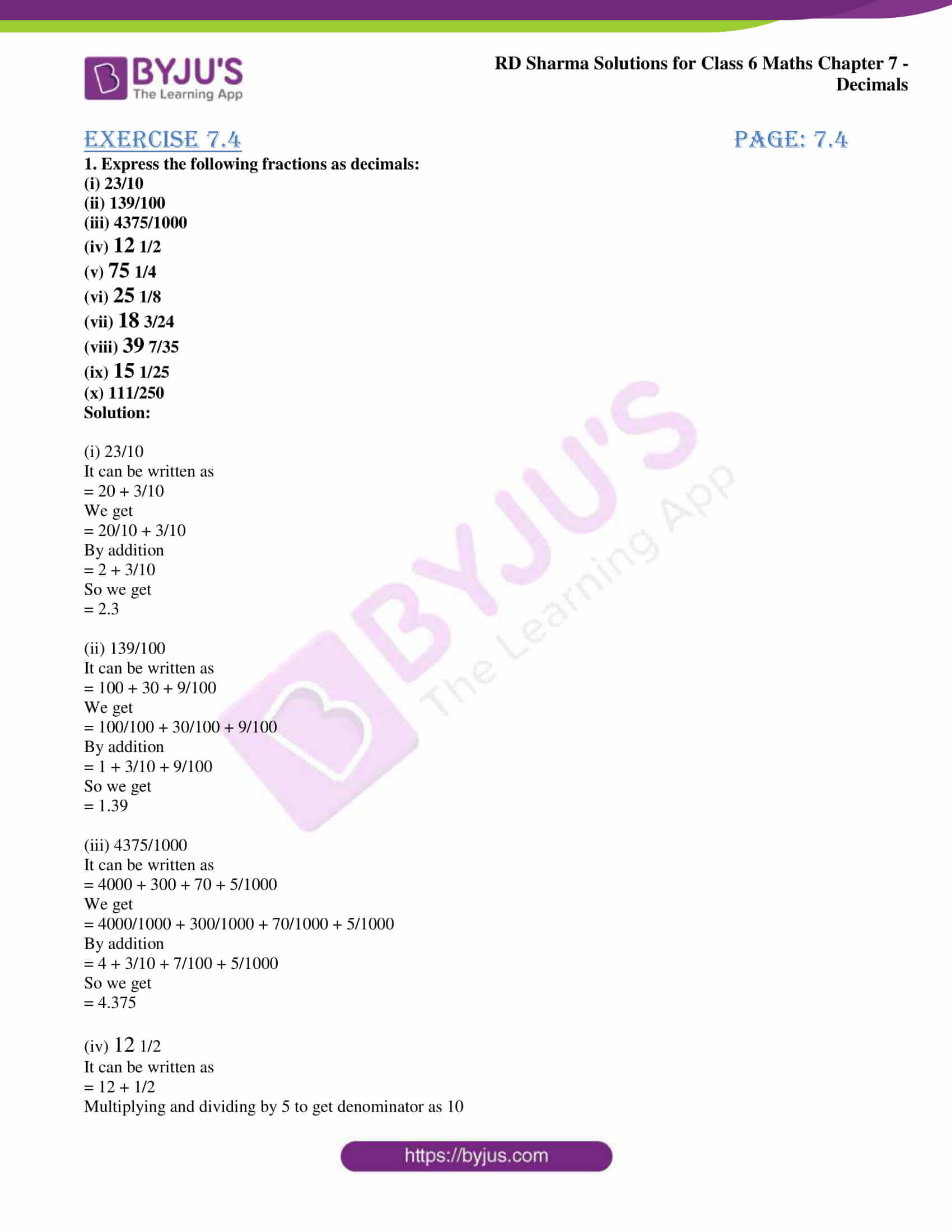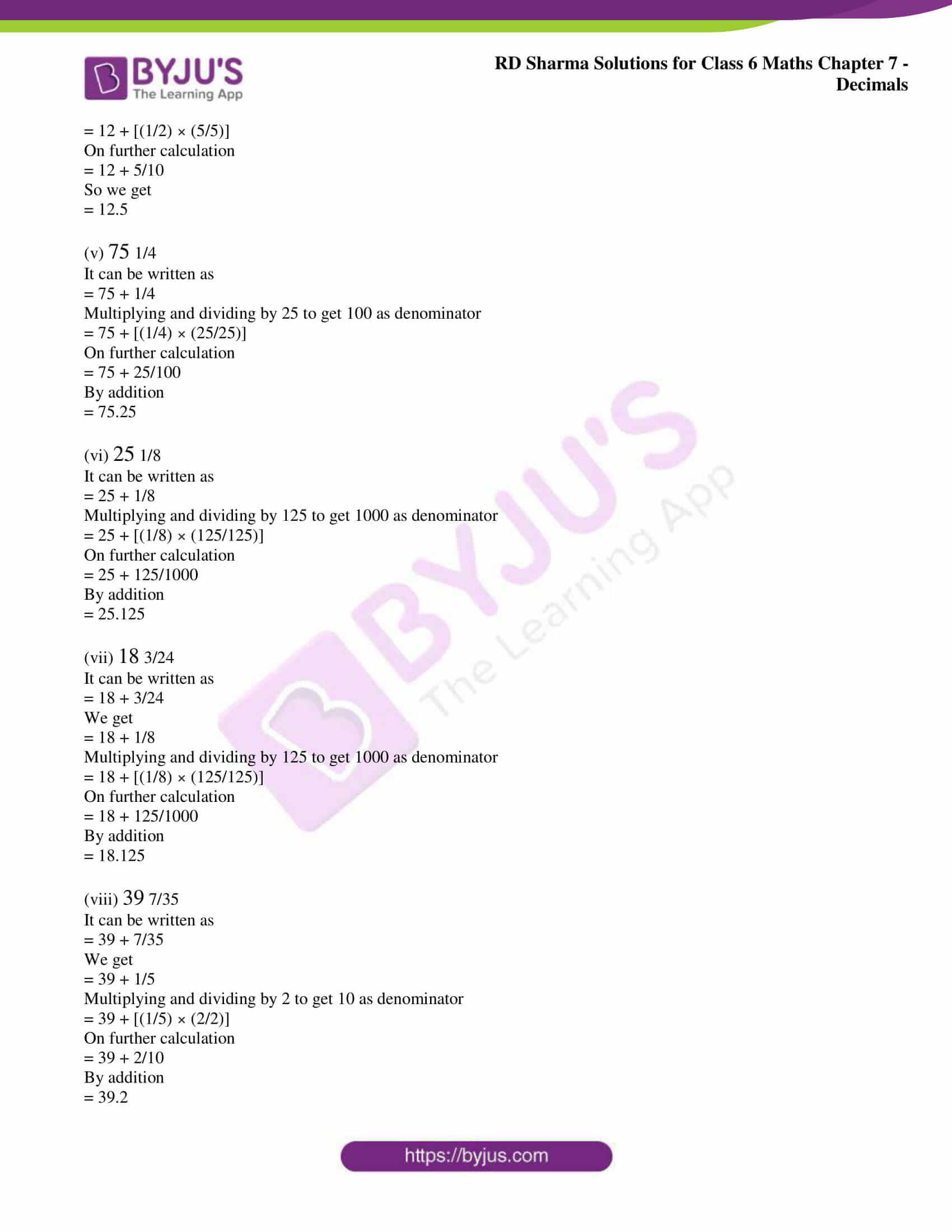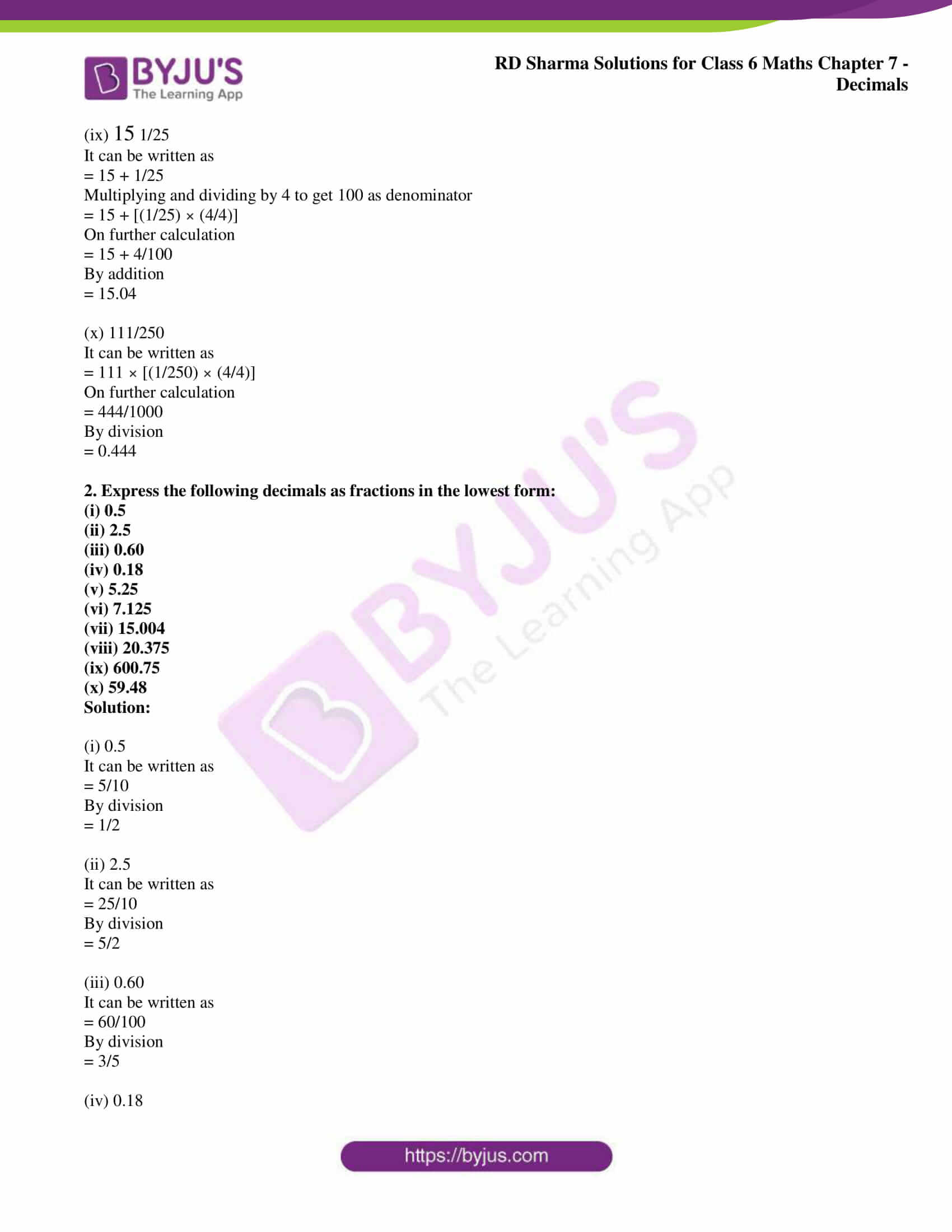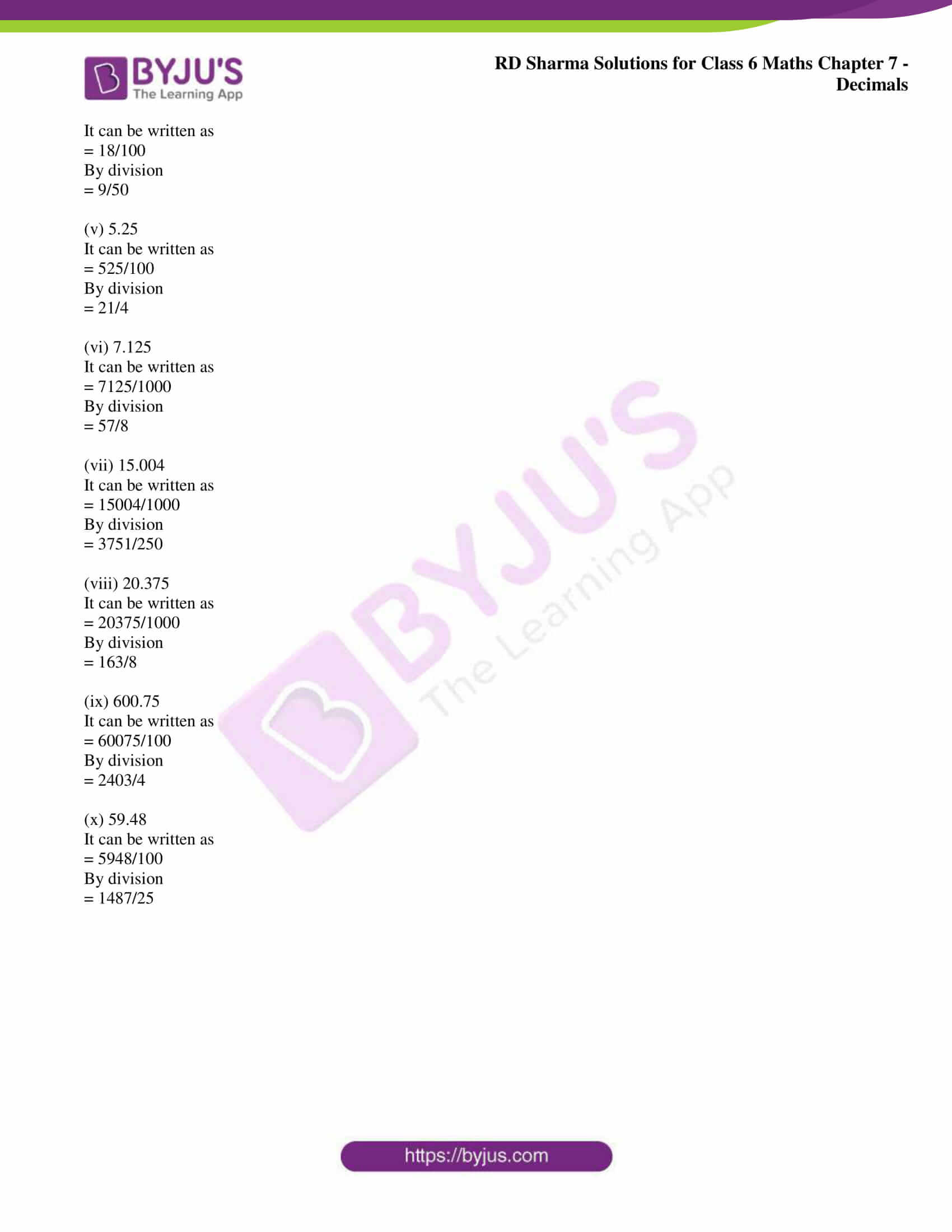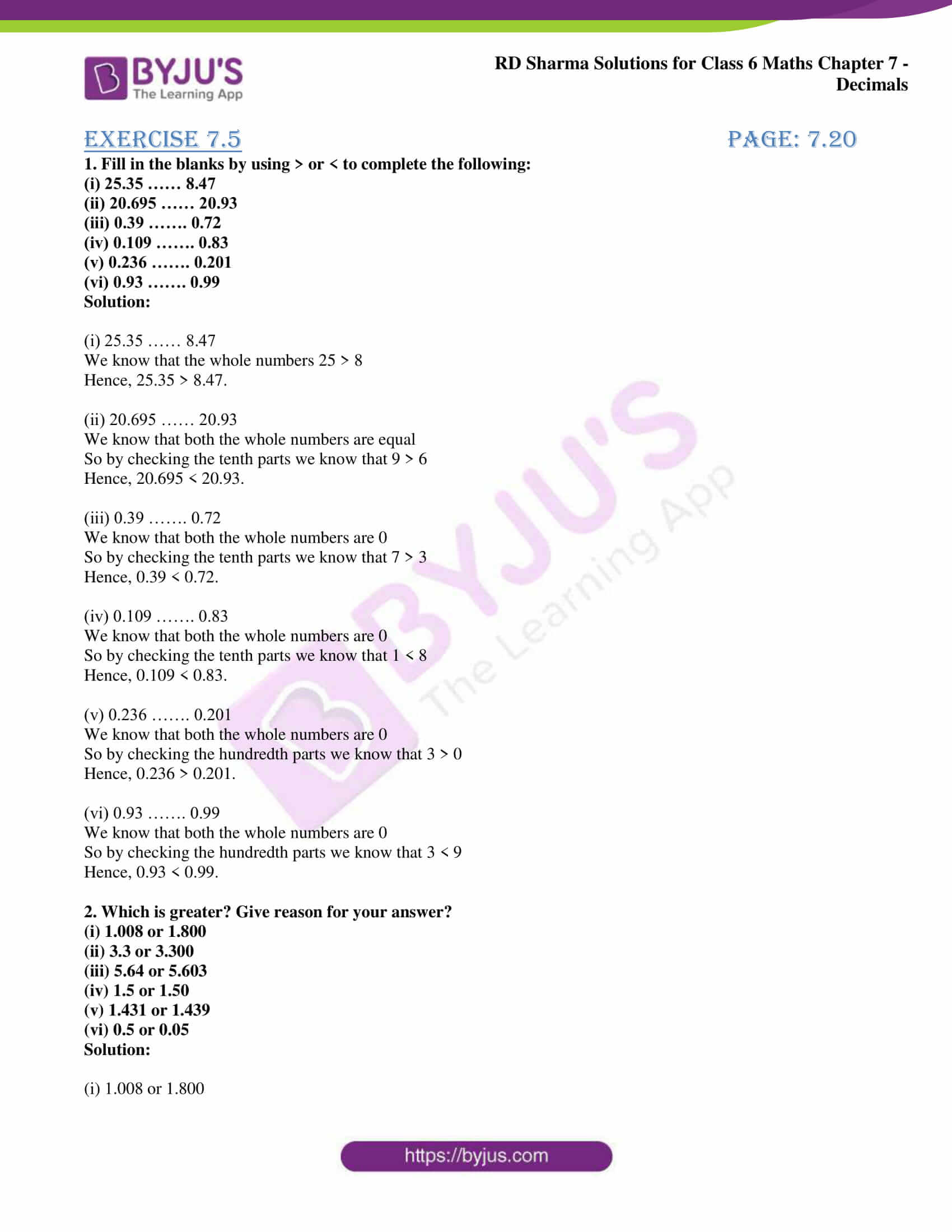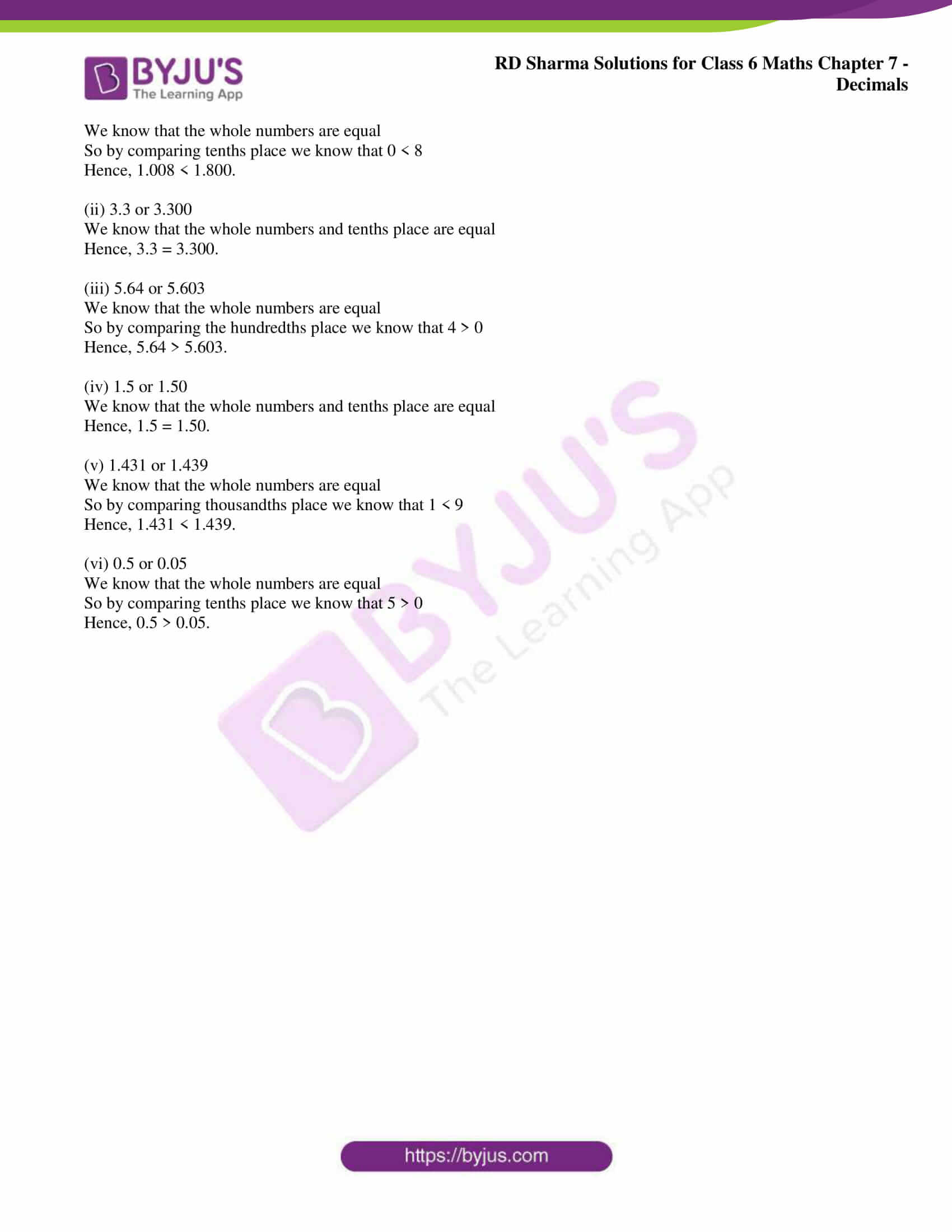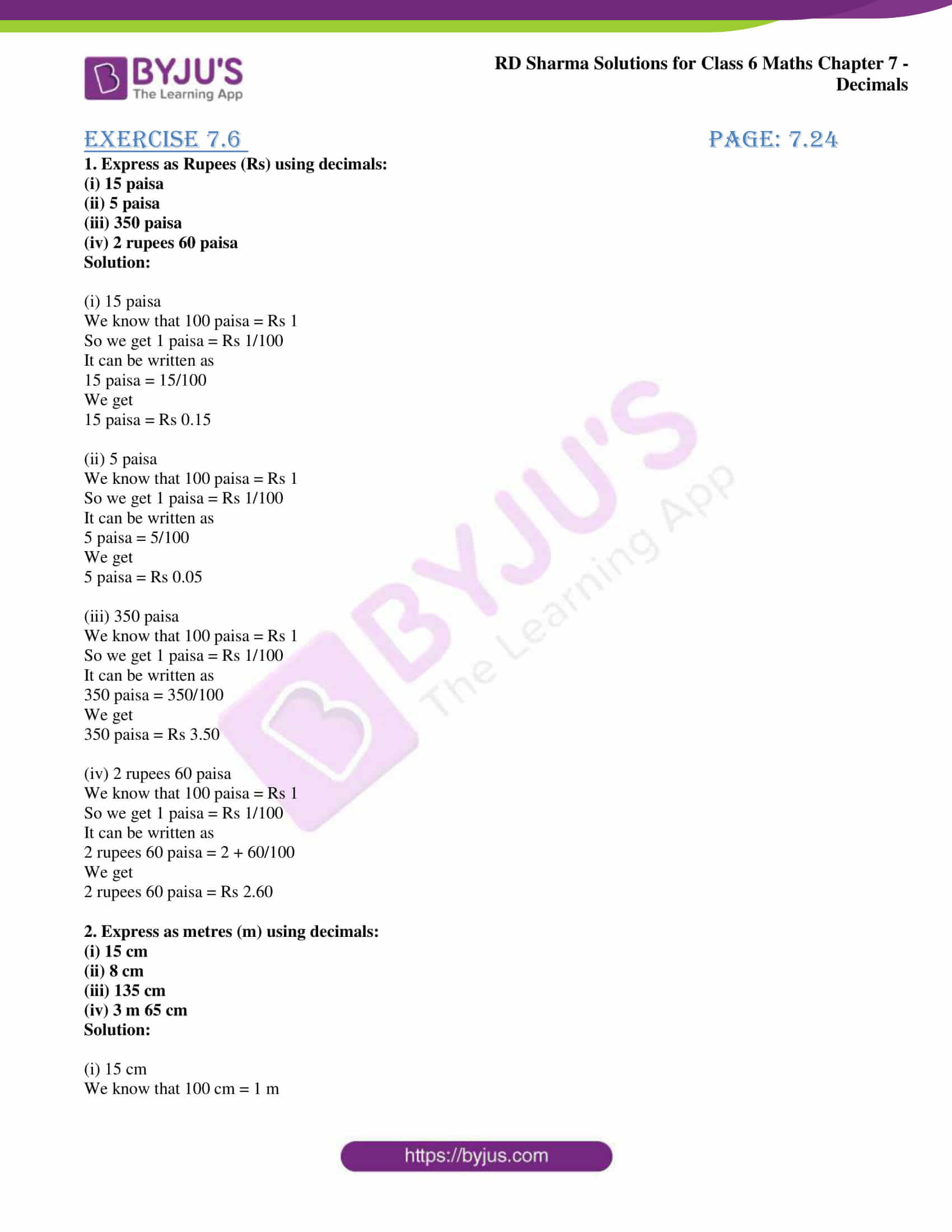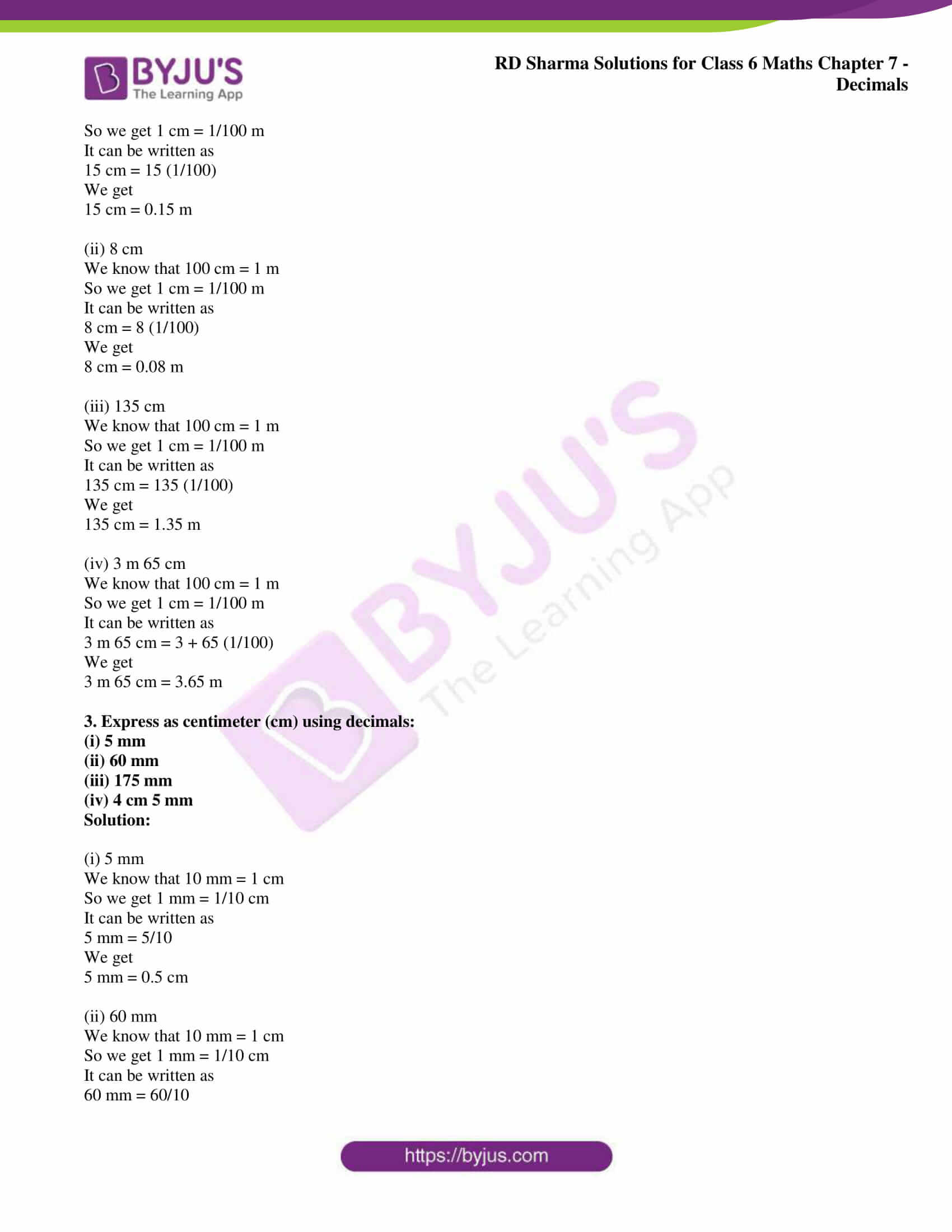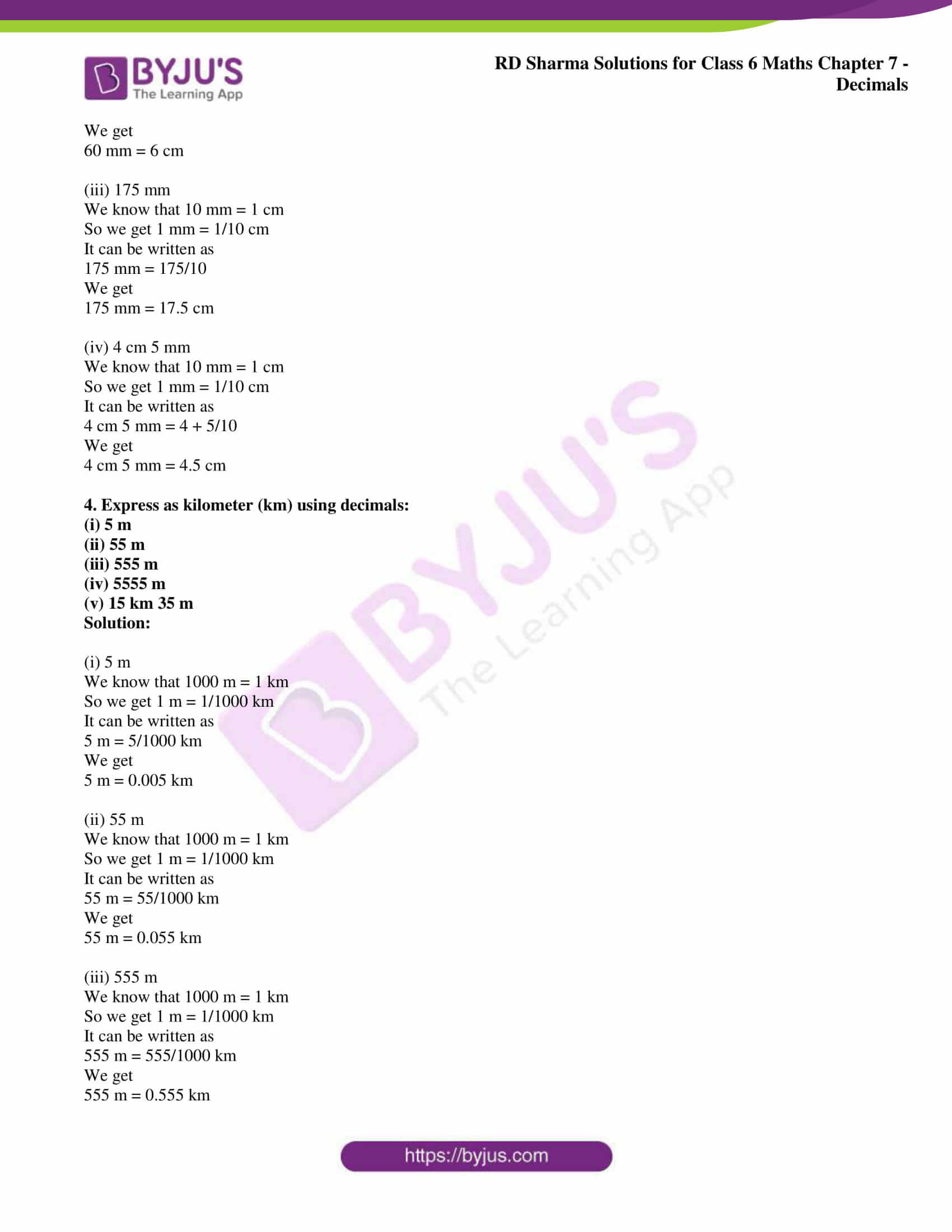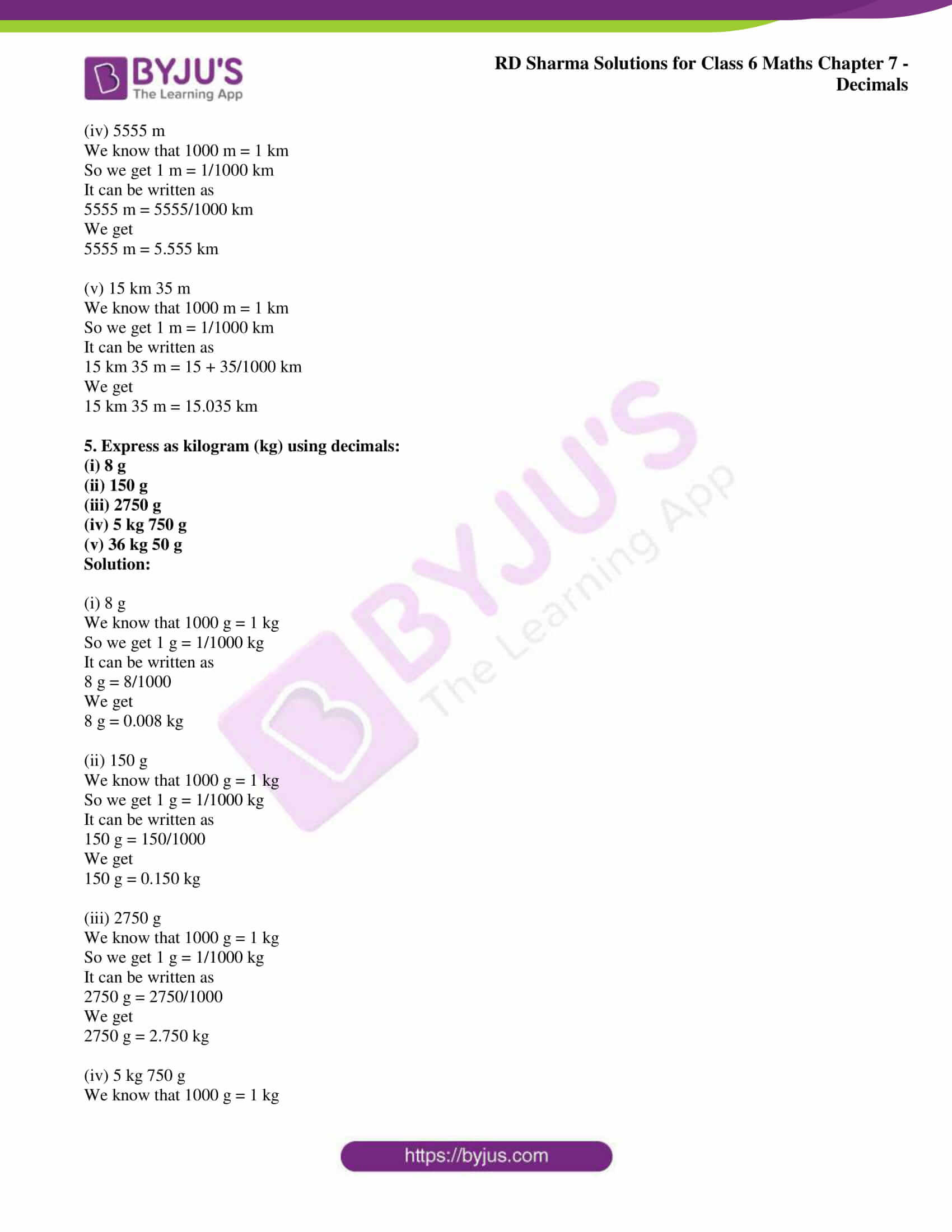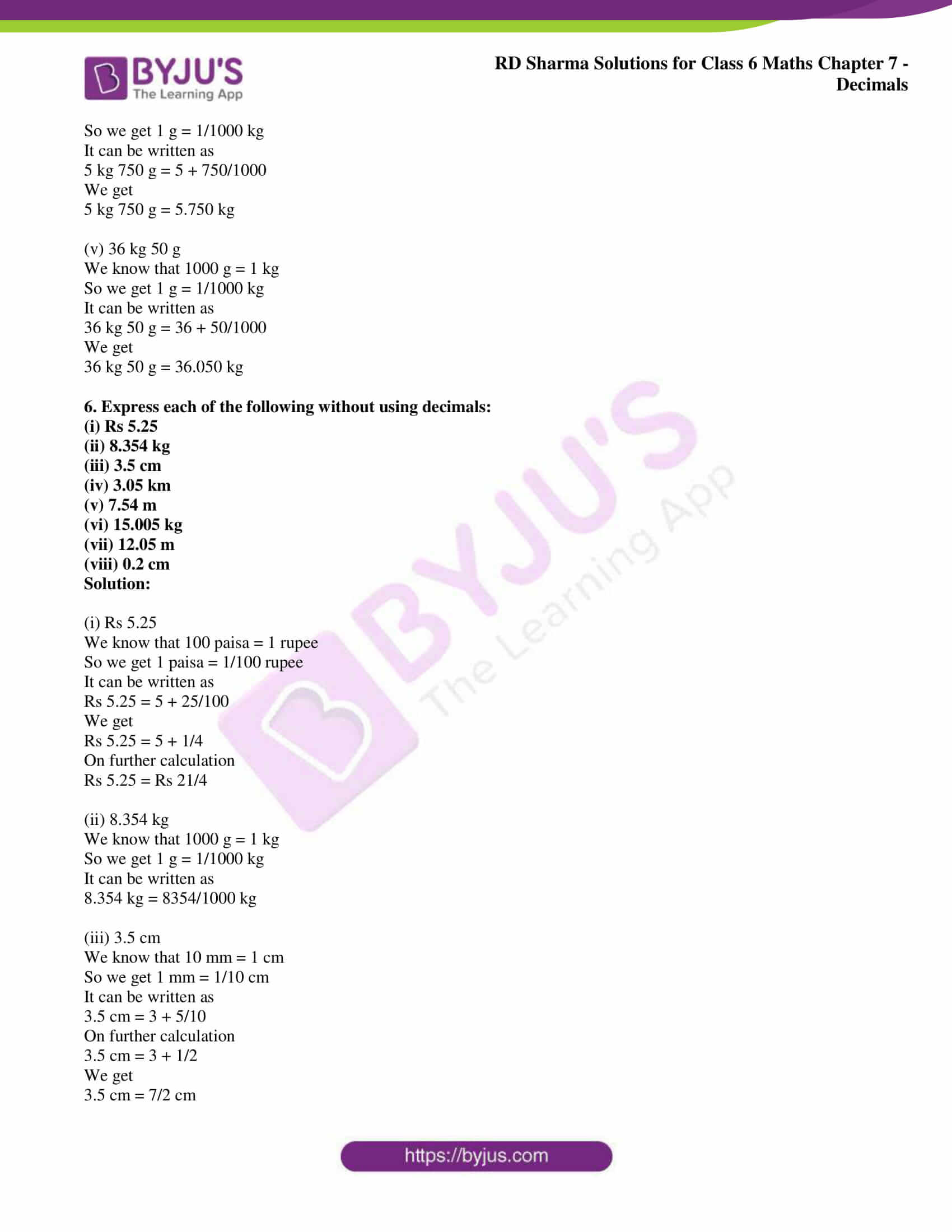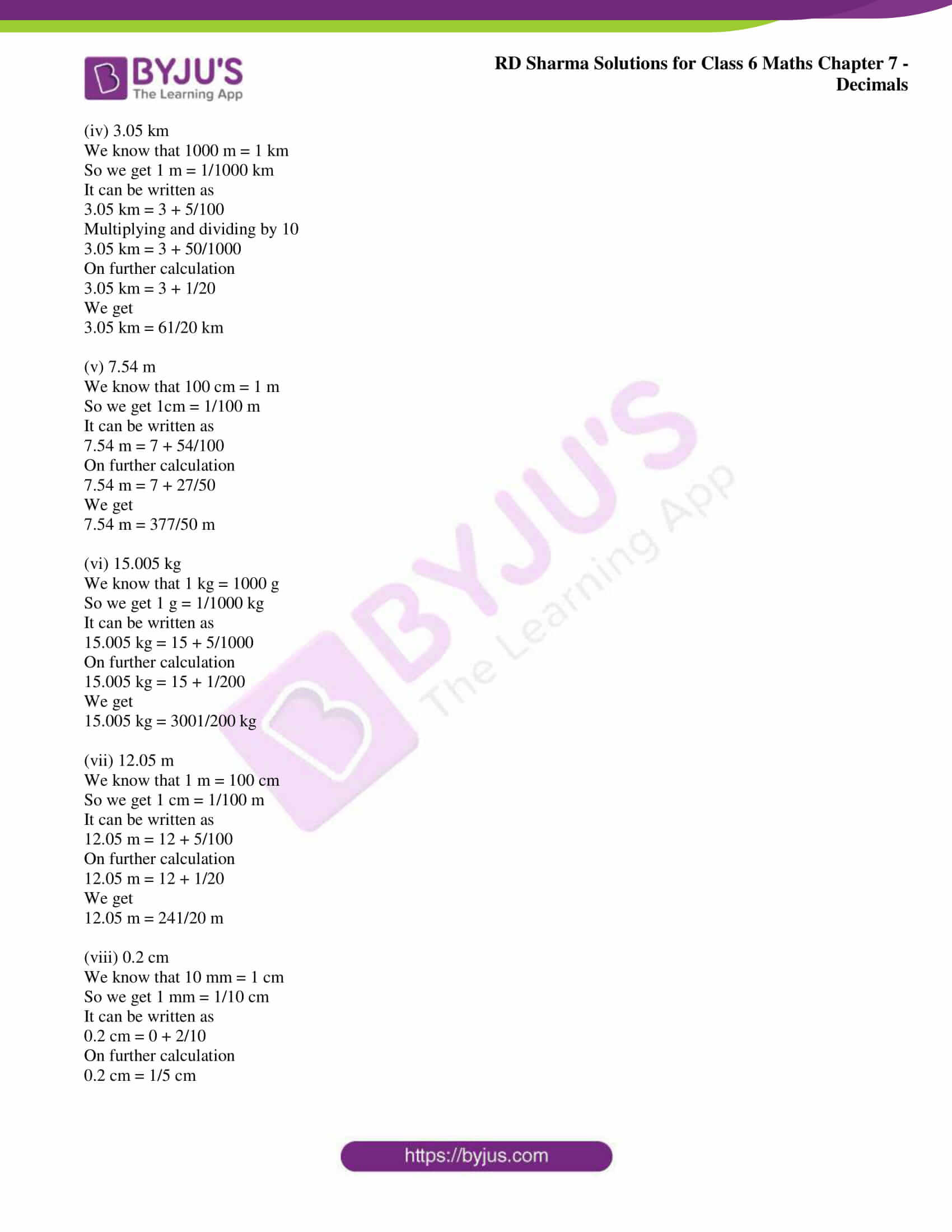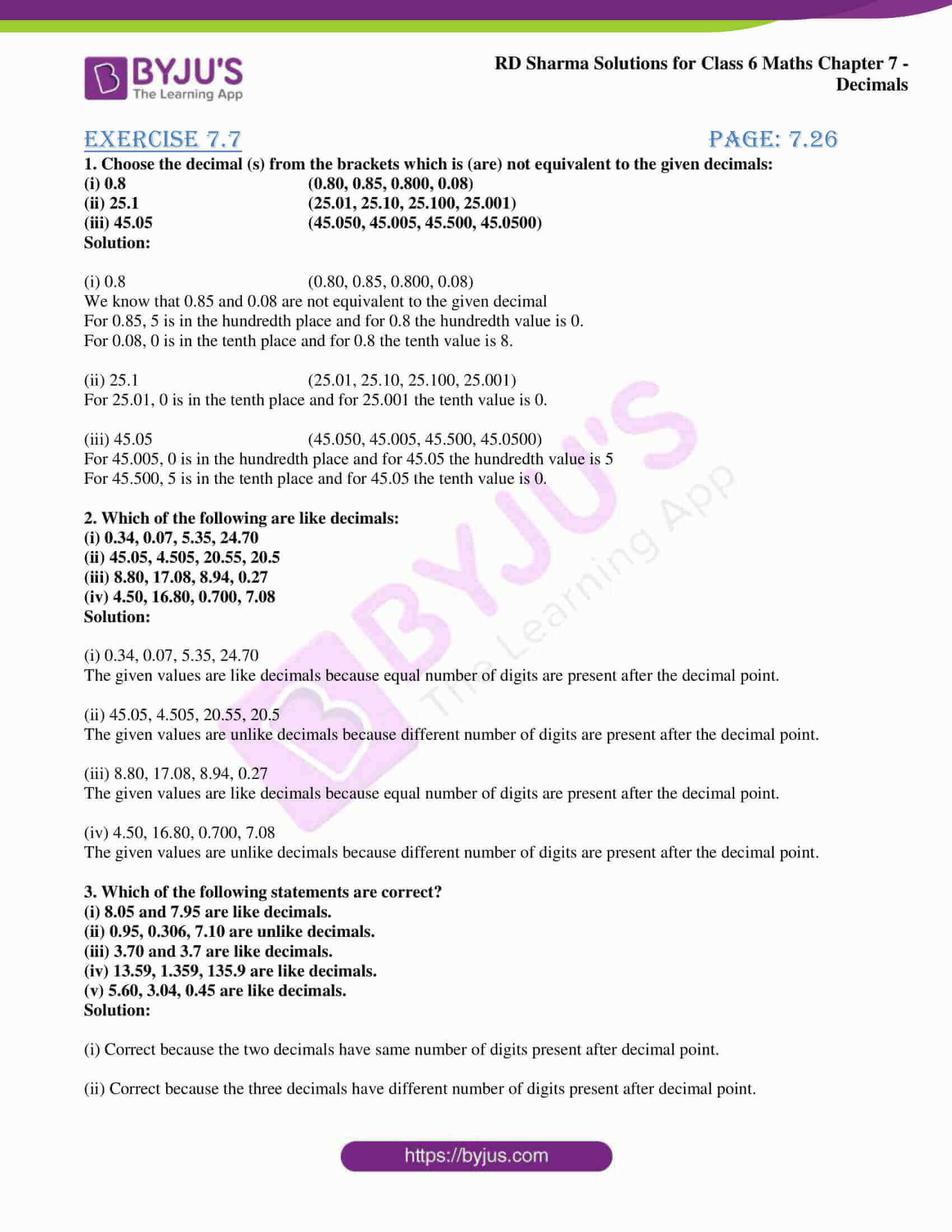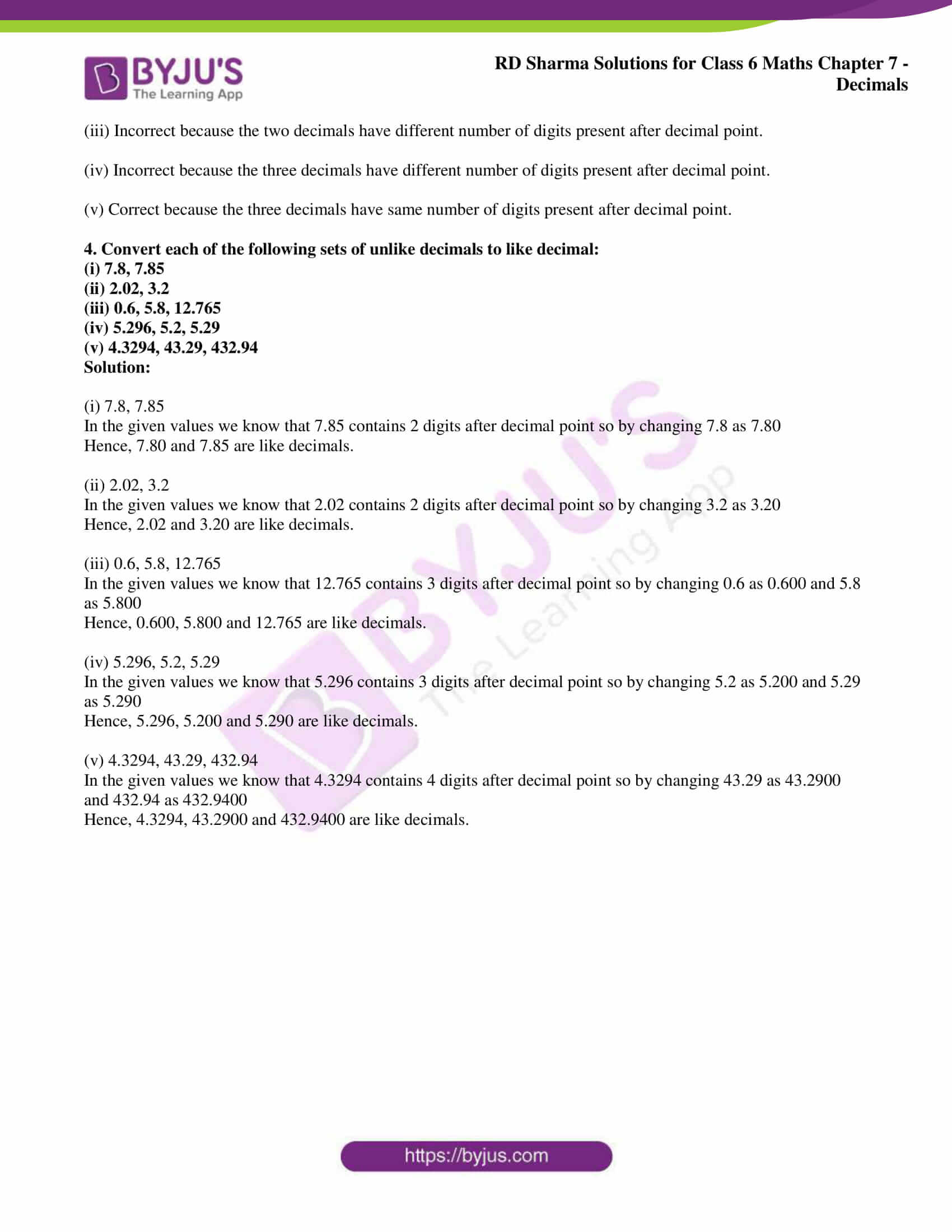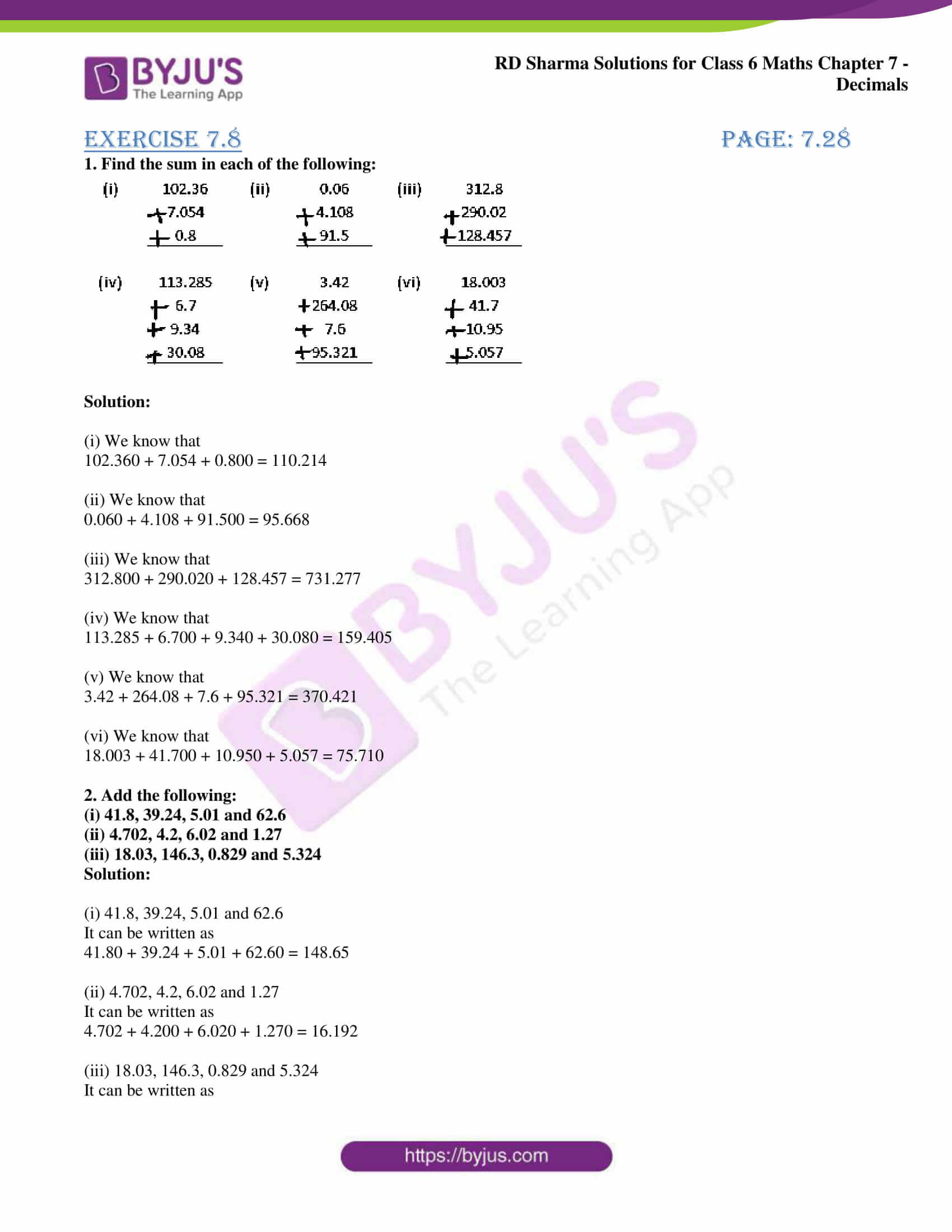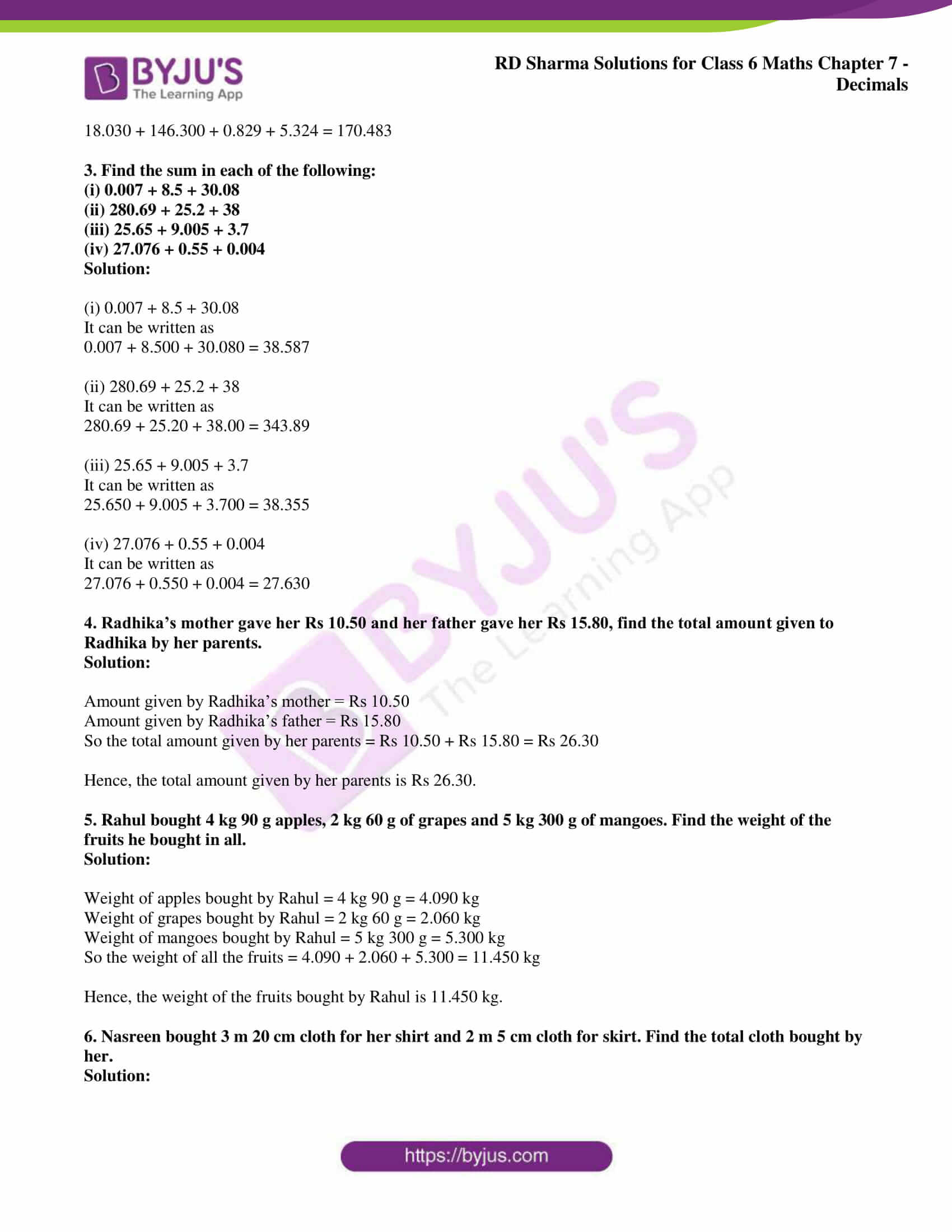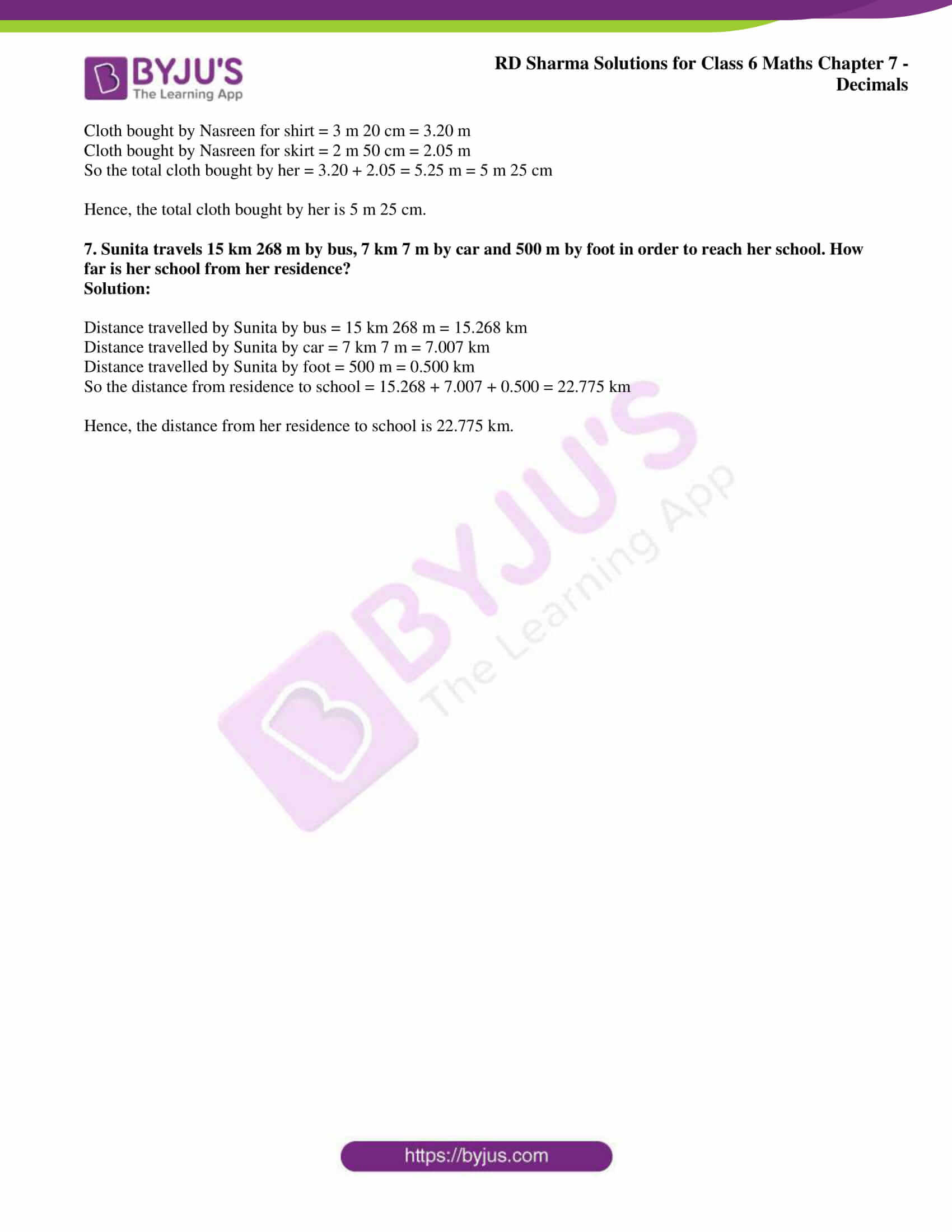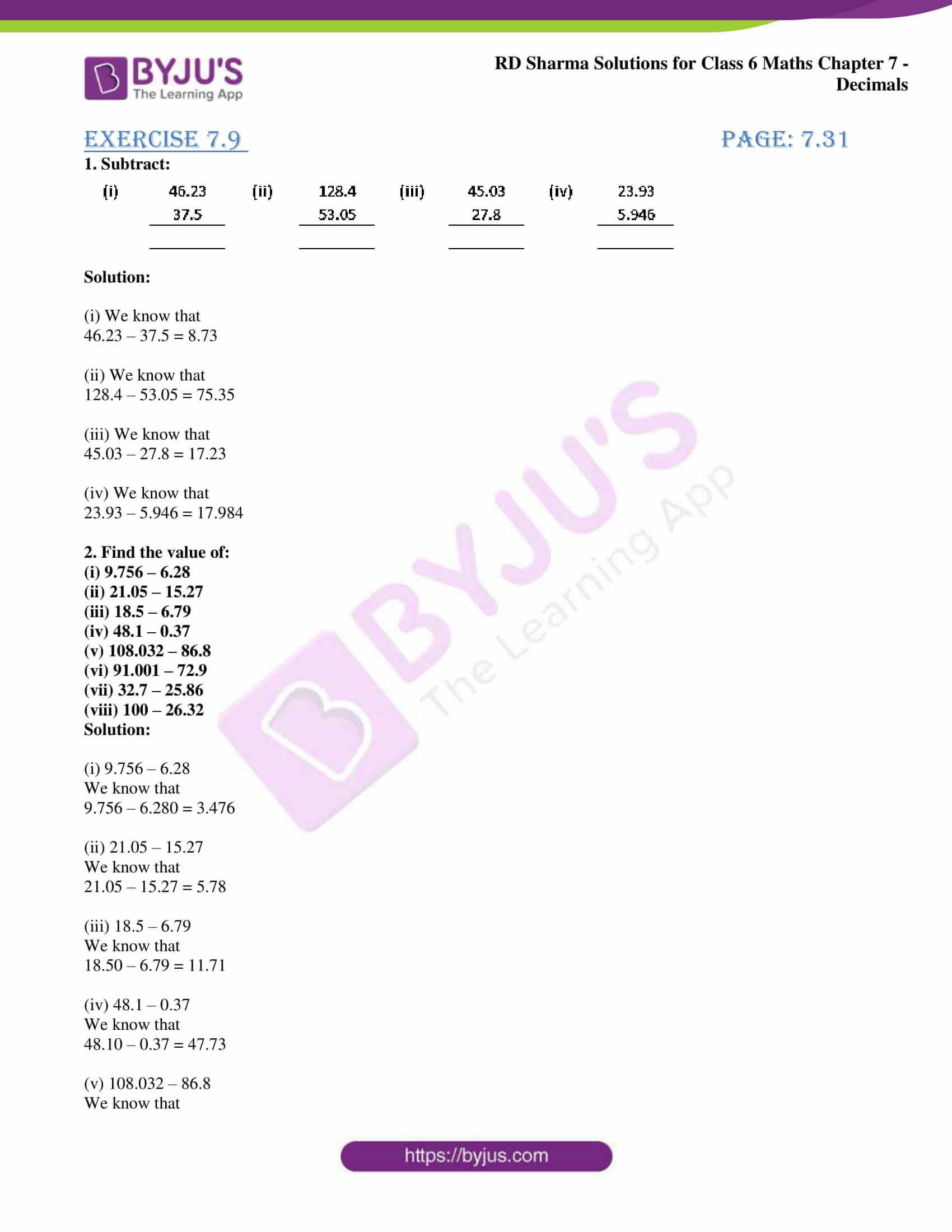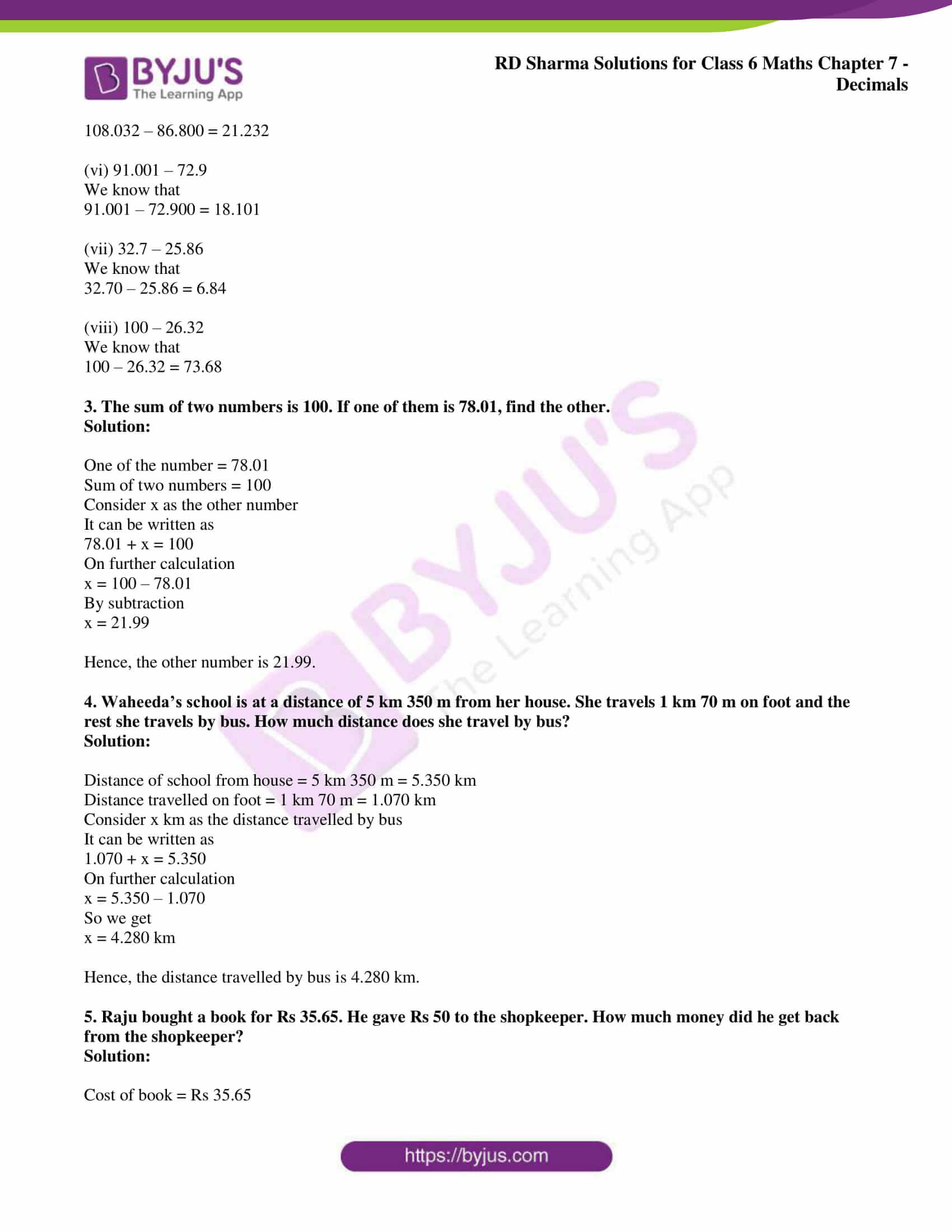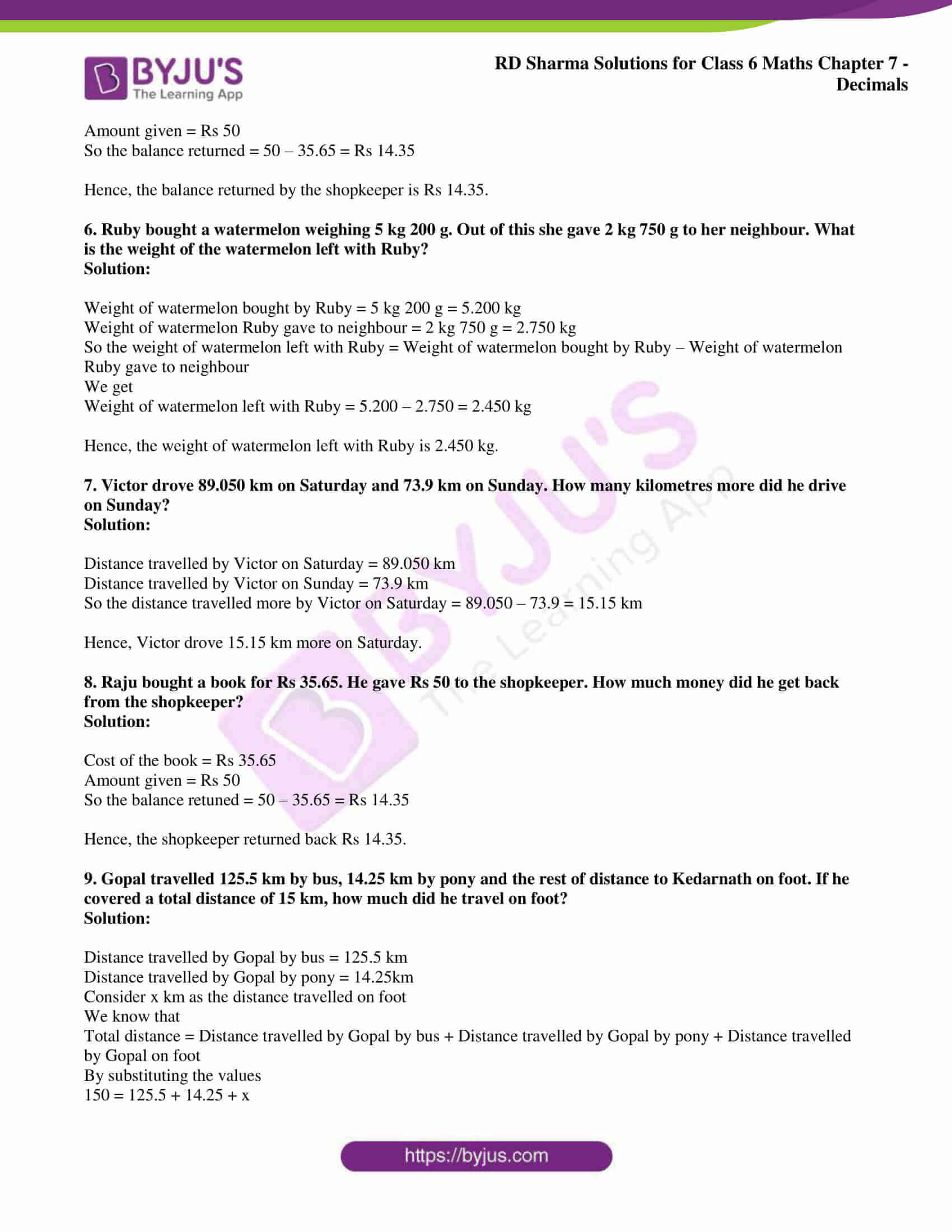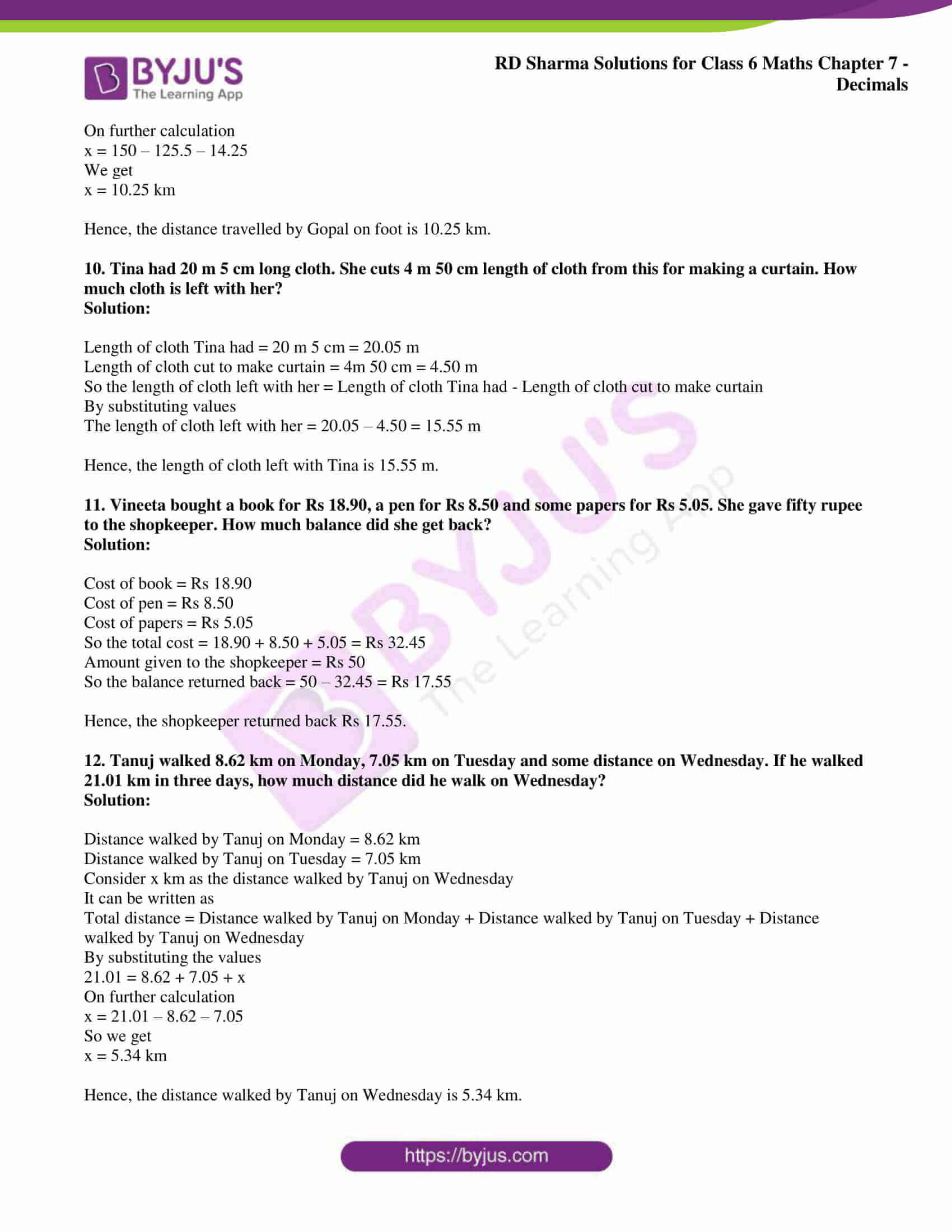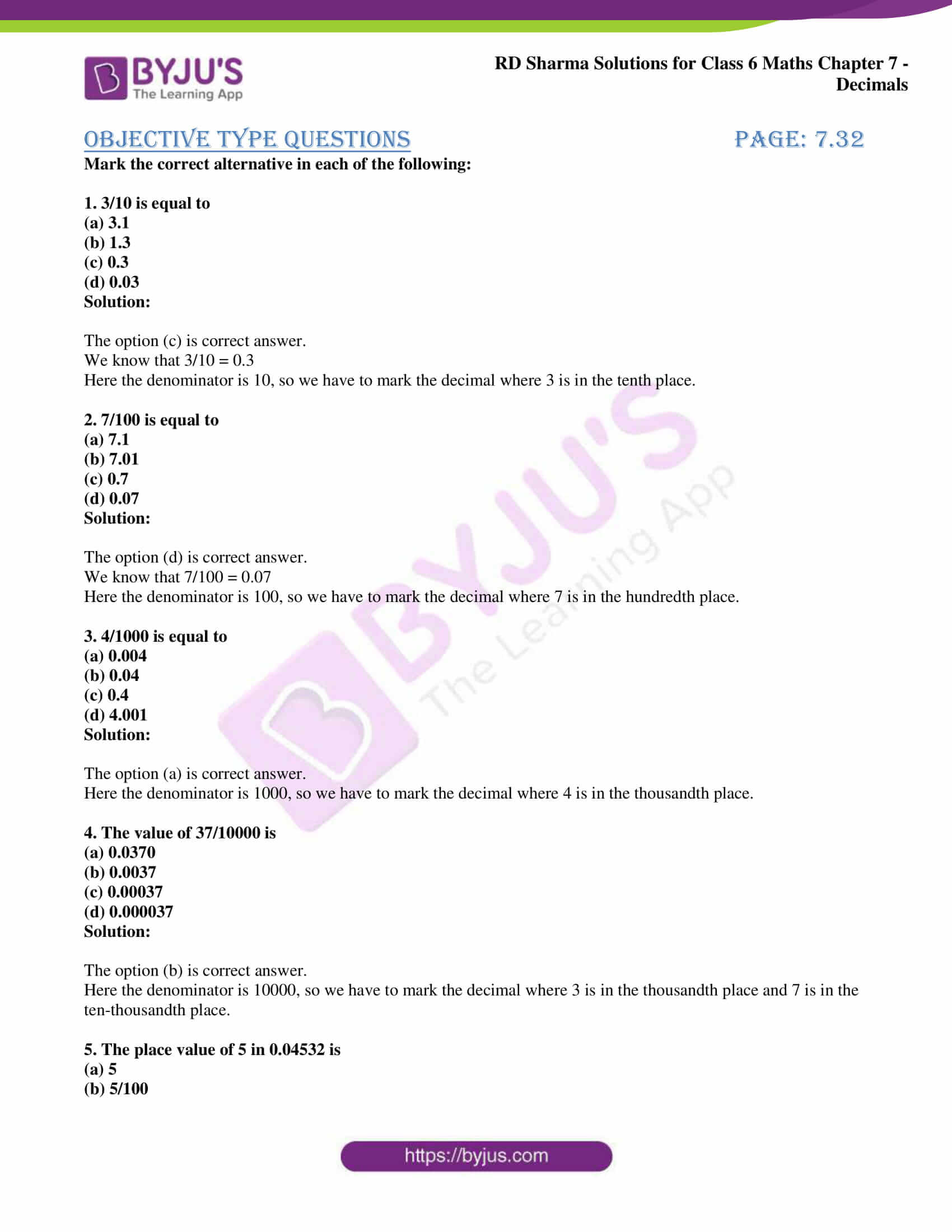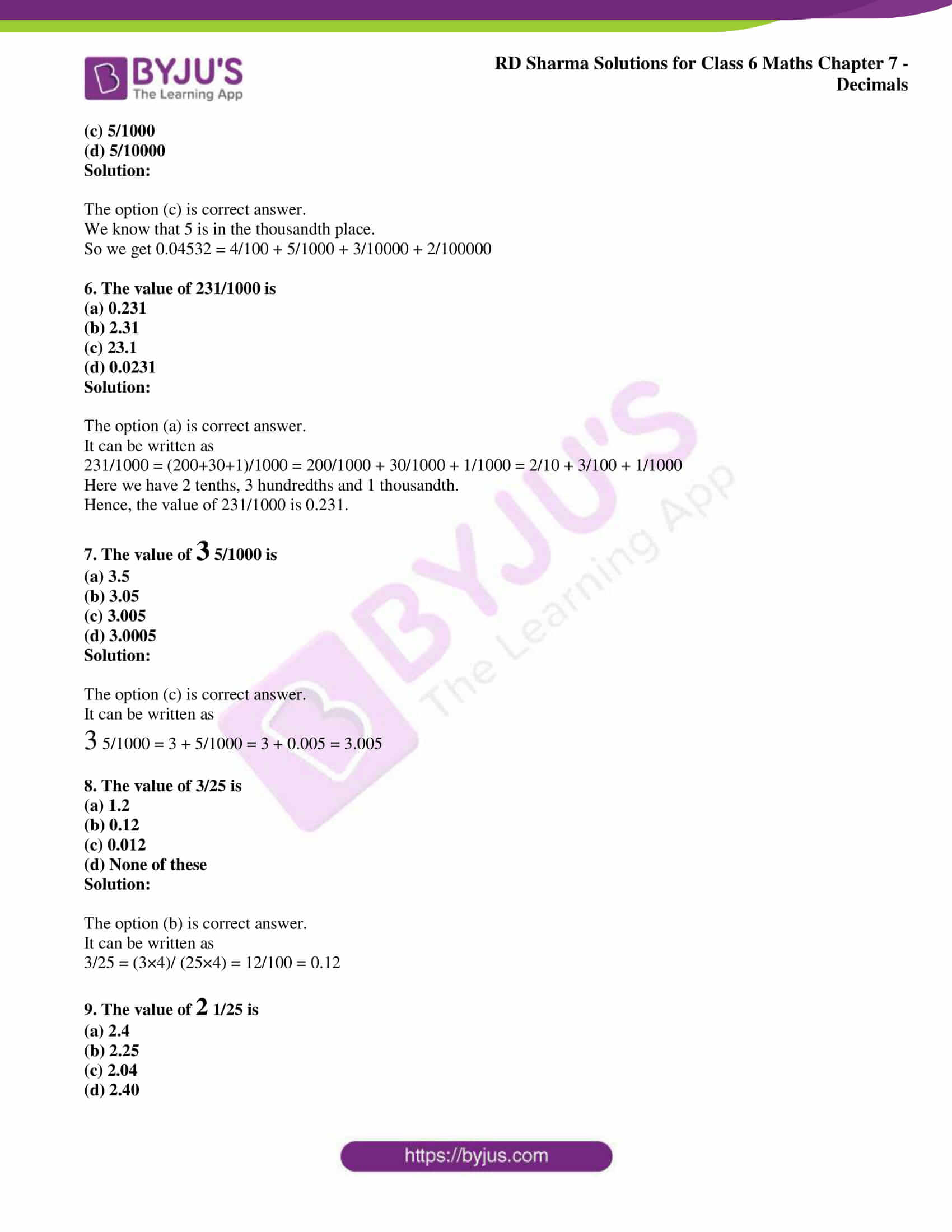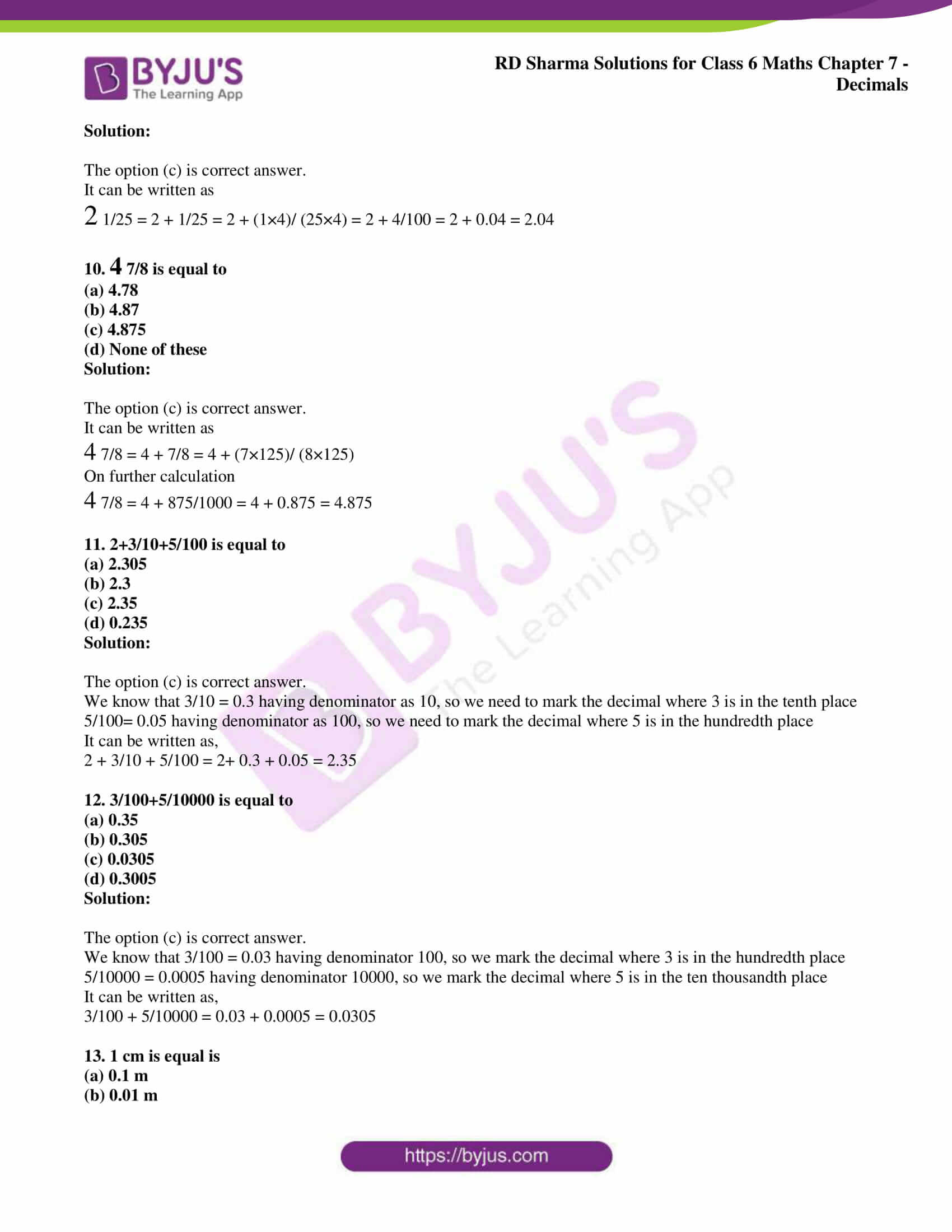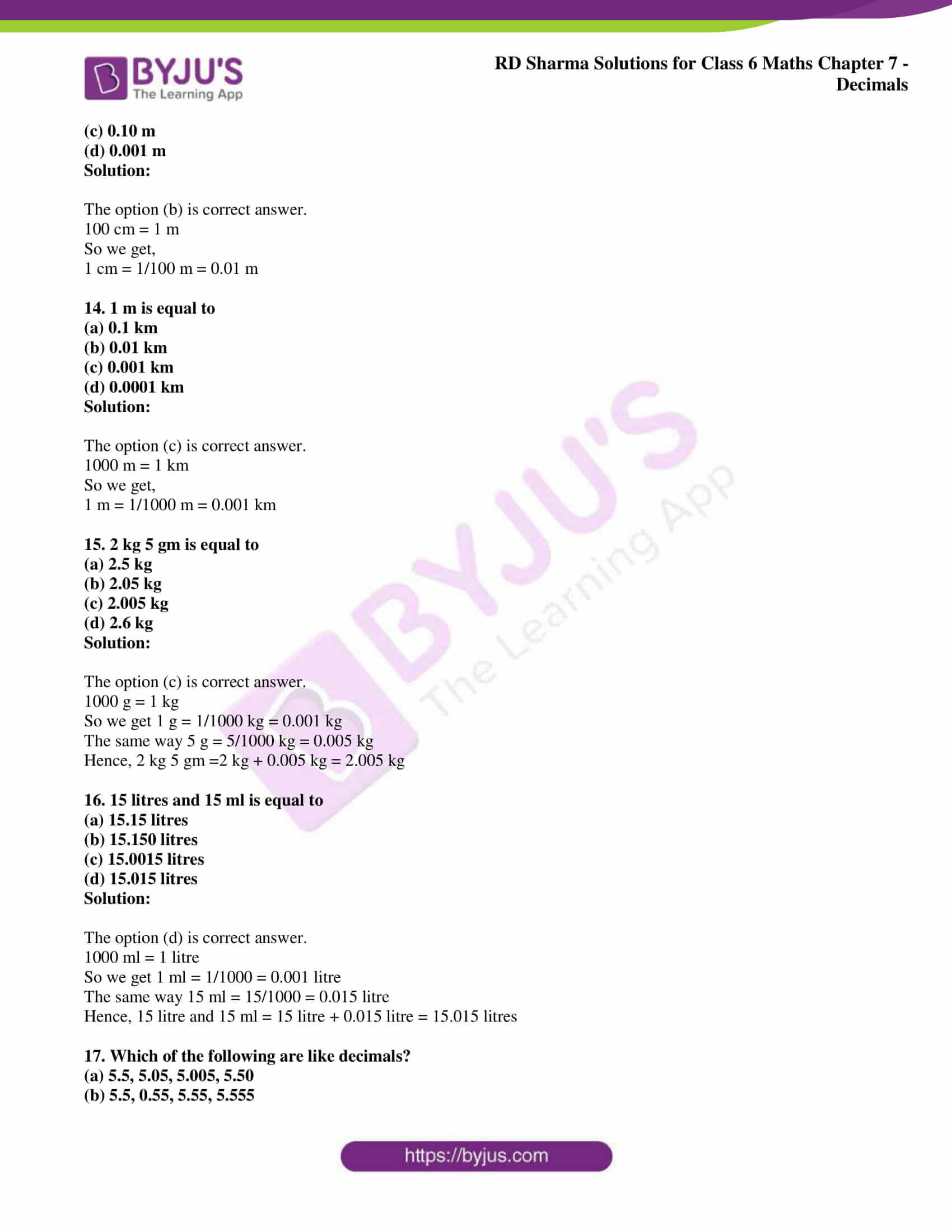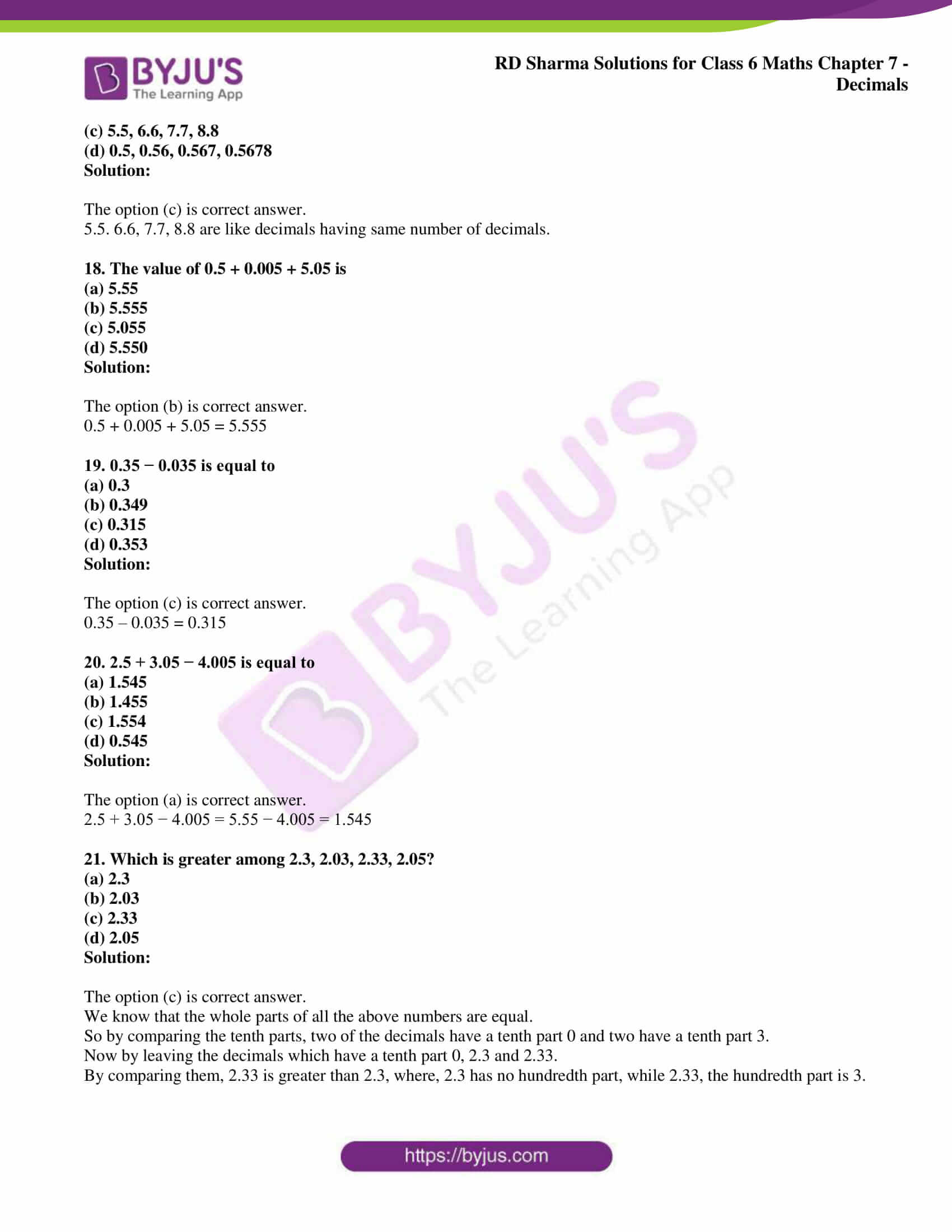## Access answers to Maths RD Sharma Solutions for Class 6 Chapter 7: Decimals

### Exercise 7.1 page: 7.4

1. Write the following decimals in the place value table:

(i) 52.5

(ii) 12.57

(iii) 15.05

(iv) 74.059

(v) 0.503

Solution: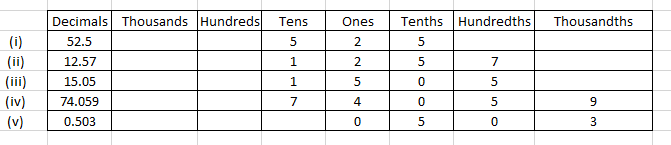2. Write the decimals shown in the following place value table: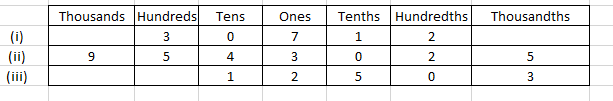Solution:

(i) We can write it as 307.12

(ii) We can write it as 9543.025

(iii) We can write it as 12.503

3. Write each of the following decimals in words:

(i) 175.04

(ii) 0.21

(iii) 9.004

(iv) 0.459

Solution:

(i) 175.04 can be written as one hundred seventy five and four hundredths.

(ii) 0.21 can be written as zero and twenty one hundredths.

(iii) 9.004 can be written as nine and four thousandths.

(iv) 0.459 can be written as zero and four hundred fifty nine thousandths.

4. Write each of the following as decimals:

(i) 65 + 2/10 + 7/100

(ii) 45 + 9/100

(iii) 88 + 5/10 + 2/1000

(iv) 3/10 + 7/1000

Solution:

(i) 65 + 2/10 + 7/100

In the above question

We know that

6 tens, 5 ones, 2 tenths and 7 hundredths

Hence, the decimal number is 65.27.

(ii) 45 + 9/100

In the above question

We know that

4 tens, 5 ones and 9 hundredths

Hence, the decimal number is 45.09.

(iii) 88 + 5/10 + 2/1000

In the above question

We know that

8 tens, 8 ones, 5 tenths and 2 thousandths

Hence, the decimal number is 88.502.

(iv) 3/10 + 7/1000

In the above question

We know that

3 tenths and 7 thousandths

Hence, the decimal number is 0.307.

5. Write each of the following as decimals:

(i) Five and four tenths

(ii) Twelve and four hundredths

(iii) Nine and Seven hundred five thousandths

(iv) Zero point five two six

(v) Forty seven and six thousandths

(vi) Eight thousandths

(vii) Nineteen and nineteen hundredths.

Solution:

(i) Five and four tenths

It can be written as

5 + 4/10 = 5.4

(ii) Twelve and four hundredths

It can be written as

12 + 4/100 = 12.04

(iii) Nine and Seven hundred five thousandths

It can be written as

9 + 705/1000 = 9.705

(iv) Zero point five two six

It can be written as

0.526

(v) Forty seven and six thousandths

It can be written as

47 + 6/1000 = 47.006

(vi) Eight thousandths

It can be written as

8/1000 = 0.008

(vii) Nineteen and nineteen hundredths

It can be written as

19 + 19/100 = 19.19

### Exercise 7.2 page: 7.9

1. Write each of the following as decimals:

(i) Three tenths

(ii) Two ones and five tenths

(iii) Thirty and one tenths

(iv) Twenty two and six tenths

(v) One hundred, two ones and three tenths

Solution:

(i) Three tenths

It can be written as

3/10 = 0.3

(ii) Two ones and five tenths

It can be written as

2 + 5/10 = 2.5

(iii) Thirty and one tenths

It can be written as

30 + 1/10 = 30.1

(iv) Twenty two and six tenths

It can be written as

22 + 6/10 = 22.6

(v) One hundred, two ones and three tenths

It can be written as

100 + 2 + 3/10 = 102.3

2. Write each of the following as decimals:

(i) 30 + 6 + 2/10

(ii) 700 + 5 + 7/10

(iii) 200 + 60 + 5 + 1/10

(iv) 200 + 70 + 9 + 5/10

Solution:

(i) 30 + 6 + 2/10

In the above question

We know that

3 tens, 6 ones and 2 tenths

Hence, the decimal is 36.2.

(ii) 700 + 5 + 7/10

In the above question

We know that

7 hundreds, 5 ones and 7 tenths

Hence, the decimal is 705.7.

(iii) 200 + 60 + 5 + 1/10

In the above question

We know that

2 hundreds, 6 tens, 5 ones and 1 tenths.

Hence, the decimal is 265.1.

(iv) 200 + 70 + 9 + 5/10

In the above question

We know that

2 hundreds, 7 tens, 9 ones and 5 tenths

Hence, the decimal is 279.5.

3. Write each of the following as decimals:

(i) 22/10

(ii) 3/2

(iii) 2/5

Solution:

(i) 22/10

Here the denominator is ten

Hence, the decimal is 2.2

(ii) 3/2

Multiplying the fraction by 5

We get

(3/2) × (5/5) = 15/10 = 1.5

(iii) 2/5

Multiplying the fraction by 2

We get

(2/5) × (2/2) = 4/10 = 0.4

4. Write each of the following as decimals:

(i) 40 2/5

(ii) 39 2/10

(iii) 4 3/5

(iv) 25 1/2

Solution:

(i) 40 2/5

In order to write in decimal we should make the denominator 10

So we get

40 + [(2/5) × (2/2)] = 40 + 4/10 = 40.4

(ii) 39 2/10

It can be written as

39 + 2/10 = 39 + 0.2 = 39.2

(iii) 4 3/5

In order to write in decimal we should make the denominator 10

So we get

4 + [(3/5) × (2/2)] = 4 + 6/10 = 4.6

(iv) 25 1/2

In order to write in decimal we should make the denominator 10

So we get

25+ [(1/2) × (5/5)] = 25 + 5/10 = 25.5

5. Write the following decimals as fractions. Reduce the fractions to lowest form:

(i) 3.8

(ii) 21.2

(iii) 6.4

(iv) 1.0

Solution:

(i) 3.8

It can be written as

= 3 + 8 tenths

On further calculation

= 3 + 8/10

We get

= 3(10/10) + 8/10

By further simplification

= 30/10 + 8/10

= 38/10

So we get

= 19/5

(ii) 21.2

It can be written as

= 21 + 2 tenths

On further calculation

= 21 + 2/10

We get

= 21(10/10) + 2/10

By further simplification

= 210/10 + 2/10

= 212/10

So we get

= 106/5

(iii) 6.4

It can be written as

= 6 + 4 tenths

On further calculation

= 6 + 4/10

We get

= 6(10/10) + 4/10

By further simplification

= 60/10 + 4/10

= 64/10

So we get

= 32/5

(iv) 1.0

Here, the number after decimal is zero so the fraction is 1.

6. Represent the following decimal numbers on the number line:

(i) 0.2

(ii) 1.9

(iii) 1.1

(iv) 2.5

Solution:

(i) 0.2 can be represented on the number line as given below: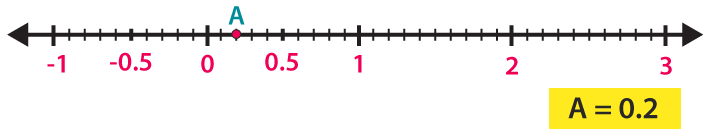(ii) 1.9 can be represented on the number line as given below: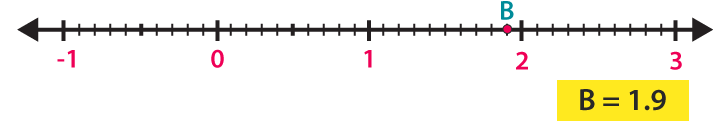(iii) 1.1 can be represented on the number line as given below: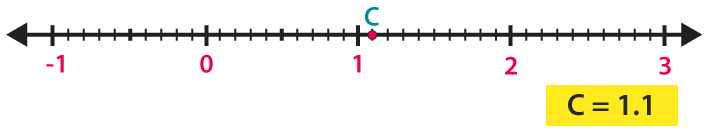(iv) 2.5 can be represented on the number line as given below: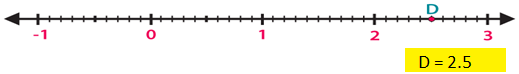7. Between which two whole numbers on the number line are the given numbers? Which one is nearer the number?

(i) 0.8

(ii) 5.1

(iii) 2.6

(iv) 6.4

(v) 9.0

(vi) 4.9

Solution:

(i) We know that

0.8 is 8 units from 0 and 2 units from 1

Hence, it is nearer to 1.

(ii) We know that

5.1 is 1 unit from 5 and 9 units from 6

Hence, it is nearer to 5.

(iii) We know that

2.6 is 6 units from 2 and 4 units from 3

Hence, it is nearer to 3.

(iv) We know that

6.4 is 4 units from 6 and 6 units from 7

Hence, it is nearer to 6.

(v) We know that

9.0 is a whole number

Hence, it is nearer to 9.

(vi) We know that

4.9 is 9 units from 4 and 1 unit from 5

Hence, it is nearer to 5.

8. Write the decimal number represented by the points on the given number line: A, B, C, D.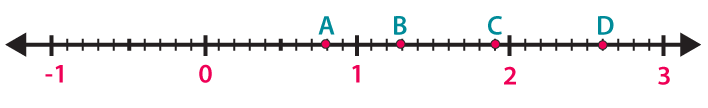Solution:

A – We know that A is at eighth place between the numbers 0 and 1

Hence, the decimal is 0.8

B – We know that B is at third place between the numbers 1 and 2

Hence, the decimal is 1.3

C – We know that C is at second place between the numbers 2 and 3

Hence, the decimal is 1.9

D – We know that D is at ninth place between the numbers 2 and 3

Hence, the decimal is 2.6

### Exercise 7.3 page: 7.14

1. Write each of the following as decimals:

(i) Five hundred twenty five and forty hundredths.

(ii) Twelve and thirty five thousandths

(iii) Fifteen and seventeen thousandths

(iv) Eighty eight and forty eight hundredths

Solution:

(i) Five hundred twenty five and forty hundredths

It can be written as

525 + 40/100 = 525.40

(ii) Twelve and thirty five thousandths

It can be written as

12 + 35/1000 = 12.035

(iii) Fifteen and seventeen thousandths

It can be written as

15 + 17/1000 = 15.017

(iv) Eighty eight and forty eight hundredths

It can be written as

88 + 48/100 = 88.48

2. Write each of the following as decimals:

(i) 137 + 5/100

(ii) 20 + 9 + 4/100

Solution:

(i) 137 + 5/100

We know that

1 hundred, 3 tens, 7 ones and 5 hundredths

Hence, the decimal is 137.05.

(ii) 20 + 9 + 4/100

We know that

2 tens, 9 ones and 4 hundredths

Hence, the decimal is 29.04.

3. Write each of the following as decimals:

(i) 8/100

(ii) 300/1000

(iii) 18/1000

(iv) 208/100

(v) 888/1000

Solution:

(i) 8/100

We know that 8/100 = 0.08

Hence, the decimal is 0.08.

(ii) 300/1000

We know that 300/1000 = 3/10

So 3 tenths

Hence, the decimal is 0.3.

(iii) 18/1000

We know that 18/1000 = 0.018

Hence, the decimal is 0.018.

(iv) 208/100

We know that 208/100 = 200/100 + 8/100

So 2 and 8 hundredths

Hence, the decimal is 2.08.

(v) 888/1000

We know that 888/1000 = 800/1000 + 80/1000 + 8/1000

We get

= 8/10 + 8/100 + 8/1000

So 8 tenths, 8 hundredths and 8 thousandths

Hence, the decimal is 0.888.

4. Write each of the following as decimals:

(i) 12 1/4

(ii) 7 1/8

(iii) 5 1/20

Solution:

(i) 12 1/4

It can be written as

= 12 + 1/4

In order to make denominator 100

Multiply and divide by 25

We get

= 12 + [(1/4) × (25/25)]

On further calculation

= 12 + 25/100

So we get

= 12.25

(ii) 7 1/8

It can be written as

= 7 + 1/8

In order to make denominator 1000

Multiply and divide by 125

We get

= 7 + [(1/8) × (125/125)]

On further calculation

= 7 + 125/1000

So we get

= 7.125

(iii) 5 1/20

It can be written as

= 5 + 1/20

In order to make denominator 100

Multiply and divide by 5

We get

= 5 + [(1/20) × (5/5)]

On further calculation

= 5 + 5/100

So we get

= 5.05

5. Write each of the following decimals as fractions. Reduce the fractions to lowest form:

(i) 0.04

(ii) 2.34

(iii) 0.342

(iv) 1.20

(v) 17.38

Solution:

(i) 0.04

It can be written as

= 0 + 0.04

We get

= 0 + 4 hundredths

On further calculation

= 0 + 4/100

So we get

= 1/25

(ii) 2.34

It can be written as

= 2 + 0.34

We get

= 2 + 34 hundredths

On further calculation

= 2 + 34/100

So we get

= 2 × (100/100) + 34/100

By further simplification

= 200/100 + 34/100

= 234/100

By division

= 117/50

(iii) 0.342

It can be written as

= 0 + 0.342

We get

= 0 + 342 thousandths

On further calculation

= 342/1000

So we get

= 171/500

(iv) 1.20

It can be written as

= 1 + 0.20

We get

= 1 + 20 hundredths

On further calculation

= 100/100 + 20/100

So we get

= 120/100

By division

= 6/5

(v) 17.38

It can be written as

= 17 + 0.38

We get

= 17 + 38 hundredths

On further calculation

= 17 + 38/100

So we get

= 17 × (100/100) + 38/100

By further simplification

= 1700/100 + 38/100

= 1738/100

By division

= 869/50

6. Write each of the following as decimals:

(i) 20 + 9 + 40/10 + 1/100

(ii) 30 + 4/10 + 8/100 + 3/1000

(iii) 137 + 5/100

(iv) 7/10 + 6/100 + 4/1000

(v) 23 + 2/10 + 6/1000

(vi) 700 + 20 + 5 + 9/100

Solution:

(i) 20 + 9 + 40/10 + 1/100

We know that

2 tens, 9 ones, 4 tenths and 1 hundredths

Hence, the decimal is 29.41.

(ii) 30 + 4/10 + 8/100 + 3/1000

We know that

3 tens, 4 tenths, 8 hundredths and 3 thousandths

Hence, the decimal is 30.483.

(iii) 137 + 5/100

We know that

1 hundred, 3 tens, 7 ones and 5 hundredths

Hence, the decimal is 137.05.

(iv) 7/10 + 6/100 + 4/1000

We know that

7 tenths, 6 hundredths and 4 thousandths

Hence, the decimal is 0.764.

(v) 23 + 2/10 + 6/1000

We know that

2 tens, 3 ones, 2 tenths and 6 thousandths

Hence, the decimal is 23.206.

(vi) 700 + 20 + 5 + 9/100

We know that

7 hundreds, 2 tens, 5 ones and 9 hundredths

Hence, the decimal is 725.09.

### Exercise 7.4 page: 7.4

1. Express the following fractions as decimals:

(i) 23/10

(ii) 139/100

(iii) 4375/1000

(iv) 12 1/2

(v) 75 1/4

(vi) 25 1/8

(vii) 18 3/24

(viii) 39 7/35

(ix) 15 1/25

(x) 111/250

Solution:

(i) 23/10

It can be written as

= 20 + 3/10

We get

= 20/10 + 3/10

= 2 + 3/10

So we get

= 2.3

(ii) 139/100

It can be written as

= 100 + 30 + 9/100

We get

= 100/100 + 30/100 + 9/100

= 1 + 3/10 + 9/100

So we get

= 1.39

(iii) 4375/1000

It can be written as

= 4000 + 300 + 70 + 5/1000

We get

= 4000/1000 + 300/1000 + 70/1000 + 5/1000

= 4 + 3/10 + 7/100 + 5/1000

So we get

= 4.375

(iv) 12 1/2

It can be written as

= 12 + 1/2

Multiplying and dividing by 5 to get denominator as 10

= 12 + [(1/2) × (5/5)]

On further calculation

= 12 + 5/10

So we get

= 12.5

(v) 75 1/4

It can be written as

= 75 + 1/4

Multiplying and dividing by 25 to get 100 as denominator

= 75 + [(1/4) × (25/25)]

On further calculation

= 75 + 25/100

= 75.25

(vi) 25 1/8

It can be written as

= 25 + 1/8

Multiplying and dividing by 125 to get 1000 as denominator

= 25 + [(1/8) × (125/125)]

On further calculation

= 25 + 125/1000

= 25.125

(vii) 18 3/24

It can be written as

= 18 + 3/24

We get

= 18 + 1/8

Multiplying and dividing by 125 to get 1000 as denominator

= 18 + [(1/8) × (125/125)]

On further calculation

= 18 + 125/1000

= 18.125

(viii) 39 7/35

It can be written as

= 39 + 7/35

We get

= 39 + 1/5

Multiplying and dividing by 2 to get 10 as denominator

= 39 + [(1/5) × (2/2)]

On further calculation

= 39 + 2/10

= 39.2

(ix) 15 1/25

It can be written as

= 15 + 1/25

Multiplying and dividing by 4 to get 100 as denominator

= 15 + [(1/25) × (4/4)]

On further calculation

= 15 + 4/100

= 15.04

(x) 111/250

It can be written as

= 111 × [(1/250) × (4/4)]

On further calculation

= 444/1000

By division

= 0.444

2. Express the following decimals as fractions in the lowest form:

(i) 0.5

(ii) 2.5

(iii) 0.60

(iv) 0.18

(v) 5.25

(vi) 7.125

(vii) 15.004

(viii) 20.375

(ix) 600.75

(x) 59.48

Solution:

(i) 0.5

It can be written as

= 5/10

By division

= 1/2

(ii) 2.5

It can be written as

= 25/10

By division

= 5/2

(iii) 0.60

It can be written as

= 60/100

By division

= 3/5

(iv) 0.18

It can be written as

= 18/100

By division

= 9/50

(v) 5.25

It can be written as

= 525/100

By division

= 21/4

(vi) 7.125

It can be written as

= 7125/1000

By division

= 57/8

(vii) 15.004

It can be written as

= 15004/1000

By division

= 3751/250

(viii) 20.375

It can be written as

= 20375/1000

By division

= 163/8

(ix) 600.75

It can be written as

= 60075/100

By division

= 2403/4

(x) 59.48

It can be written as

= 5948/100

By division

= 1487/25

### Exercise 7.5 page: 7.20

1. Fill in the blanks by using > or < to complete the following:

(i) 25.35 …… 8.47

(ii) 20.695 …… 20.93

(iii) 0.39 ……. 0.72

(iv) 0.109 ……. 0.83

(v) 0.236 ……. 0.201

(vi) 0.93 ……. 0.99

Solution:

(i) 25.35 …… 8.47

We know that the whole numbers 25 > 8

Hence, 25.35 > 8.47.

(ii) 20.695 …… 20.93

We know that both the whole numbers are equal

So by checking the tenth parts we know that 9 > 6

Hence, 20.695 < 20.93.

(iii) 0.39 ……. 0.72

We know that both the whole numbers are 0

So by checking the tenth parts we know that 7 > 3

Hence, 0.39 < 0.72.

(iv) 0.109 ……. 0.83

We know that both the whole numbers are 0

So by checking the tenth parts we know that 1 < 8

Hence, 0.109 < 0.83.

(v) 0.236 ……. 0.201

We know that both the whole numbers are 0

So by checking the hundredth parts we know that 3 > 0

Hence, 0.236 > 0.201.

(vi) 0.93 ……. 0.99

We know that both the whole numbers are 0

So by checking the hundredth parts we know that 3 < 9

Hence, 0.93 < 0.99.

(i) 1.008 or 1.800

(ii) 3.3 or 3.300

(iii) 5.64 or 5.603

(iv) 1.5 or 1.50

(v) 1.431 or 1.439

(vi) 0.5 or 0.05

Solution:

(i) 1.008 or 1.800

We know that the whole numbers are equal

So by comparing tenths place we know that 0 < 8

Hence, 1.008 < 1.800.

(ii) 3.3 or 3.300

We know that the whole numbers and tenths place are equal

Hence, 3.3 = 3.300.

(iii) 5.64 or 5.603

We know that the whole numbers are equal

So by comparing the hundredths place we know that 4 > 0

Hence, 5.64 > 5.603.

(iv) 1.5 or 1.50

We know that the whole numbers and tenths place are equal

Hence, 1.5 = 1.50.

(v) 1.431 or 1.439

We know that the whole numbers are equal

So by comparing thousandths place we know that 1 < 9

Hence, 1.431 < 1.439.

(vi) 0.5 or 0.05

We know that the whole numbers are equal

So by comparing tenths place we know that 5 > 0

Hence, 0.5 > 0.05.

### Exercise 7.6 page: 7.24

1. Express as Rupees (Rs) using decimals:

(i) 15 paisa

(ii) 5 paisa

(iii) 350 paisa

(iv) 2 rupees 60 paisa

Solution:

(i) 15 paisa

We know that 100 paisa = Rs 1

So we get 1 paisa = Rs 1/100

It can be written as

15 paisa = 15/100

We get

15 paisa = Rs 0.15

(ii) 5 paisa

We know that 100 paisa = Rs 1

So we get 1 paisa = Rs 1/100

It can be written as

5 paisa = 5/100

We get

5 paisa = Rs 0.05

(iii) 350 paisa

We know that 100 paisa = Rs 1

So we get 1 paisa = Rs 1/100

It can be written as

350 paisa = 350/100

We get

350 paisa = Rs 3.50

(iv) 2 rupees 60 paisa

We know that 100 paisa = Rs 1

So we get 1 paisa = Rs 1/100

It can be written as

2 rupees 60 paisa = 2 + 60/100

We get

2 rupees 60 paisa = Rs 2.60

2. Express as metres (m) using decimals:

(i) 15 cm

(ii) 8 cm

(iii) 135 cm

(iv) 3 m 65 cm

Solution:

(i) 15 cm

We know that 100 cm = 1 m

So we get 1 cm = 1/100 m

It can be written as

15 cm = 15 (1/100)

We get

15 cm = 0.15 m

(ii) 8 cm

We know that 100 cm = 1 m

So we get 1 cm = 1/100 m

It can be written as

8 cm = 8 (1/100)

We get

8 cm = 0.08 m

(iii) 135 cm

We know that 100 cm = 1 m

So we get 1 cm = 1/100 m

It can be written as

135 cm = 135 (1/100)

We get

135 cm = 1.35 m

(iv) 3 m 65 cm

We know that 100 cm = 1 m

So we get 1 cm = 1/100 m

It can be written as

3 m 65 cm = 3 + 65 (1/100)

We get

3 m 65 cm = 3.65 m

3. Express as centimeter (cm) using decimals:

(i) 5 mm

(ii) 60 mm

(iii) 175 mm

(iv) 4 cm 5 mm

Solution:

(i) 5 mm

We know that 10 mm = 1 cm

So we get 1 mm = 1/10 cm

It can be written as

5 mm = 5/10

We get

5 mm = 0.5 cm

(ii) 60 mm

We know that 10 mm = 1 cm

So we get 1 mm = 1/10 cm

It can be written as

60 mm = 60/10

We get

60 mm = 6 cm

(iii) 175 mm

We know that 10 mm = 1 cm

So we get 1 mm = 1/10 cm

It can be written as

175 mm = 175/10

We get

175 mm = 17.5 cm

(iv) 4 cm 5 mm

We know that 10 mm = 1 cm

So we get 1 mm = 1/10 cm

It can be written as

4 cm 5 mm = 4 + 5/10

We get

4 cm 5 mm = 4.5 cm

4. Express as kilometer (km) using decimals:

(i) 5 m

(ii) 55 m

(iii) 555 m

(iv) 5555 m

(v) 15 km 35 m

Solution:

(i) 5 m

We know that 1000 m = 1 km

So we get 1 m = 1/1000 km

It can be written as

5 m = 5/1000 km

We get

5 m = 0.005 km

(ii) 55 m

We know that 1000 m = 1 km

So we get 1 m = 1/1000 km

It can be written as

55 m = 55/1000 km

We get

55 m = 0.055 km

(iii) 555 m

We know that 1000 m = 1 km

So we get 1 m = 1/1000 km

It can be written as

555 m = 555/1000 km

We get

555 m = 0.555 km

(iv) 5555 m

We know that 1000 m = 1 km

So we get 1 m = 1/1000 km

It can be written as

5555 m = 5555/1000 km

We get

5555 m = 5.555 km

(v) 15 km 35 m

We know that 1000 m = 1 km

So we get 1 m = 1/1000 km

It can be written as

15 km 35 m = 15 + 35/1000 km

We get

15 km 35 m = 15.035 km

5. Express as kilogram (kg) using decimals:

(i) 8 g

(ii) 150 g

(iii) 2750 g

(iv) 5 kg 750 g

(v) 36 kg 50 g

Solution:

(i) 8 g

We know that 1000 g = 1 kg

So we get 1 g = 1/1000 kg

It can be written as

8 g = 8/1000

We get

8 g = 0.008 kg

(ii) 150 g

We know that 1000 g = 1 kg

So we get 1 g = 1/1000 kg

It can be written as

150 g = 150/1000

We get

150 g = 0.150 kg

(iii) 2750 g

We know that 1000 g = 1 kg

So we get 1 g = 1/1000 kg

It can be written as

2750 g = 2750/1000

We get

2750 g = 2.750 kg

(iv) 5 kg 750 g

We know that 1000 g = 1 kg

So we get 1 g = 1/1000 kg

It can be written as

5 kg 750 g = 5 + 750/1000

We get

5 kg 750 g = 5.750 kg

(v) 36 kg 50 g

We know that 1000 g = 1 kg

So we get 1 g = 1/1000 kg

It can be written as

36 kg 50 g = 36 + 50/1000

We get

36 kg 50 g = 36.050 kg

6. Express each of the following without using decimals:

(i) Rs 5.25

(ii) 8.354 kg

(iii) 3.5 cm

(iv) 3.05 km

(v) 7.54 m

(vi) 15.005 kg

(vii) 12.05 m

(viii) 0.2 cm

Solution:

(i) Rs 5.25

We know that 100 paisa = 1 rupee

So we get 1 paisa = 1/100 rupee

It can be written as

Rs 5.25 = 5 + 25/100

We get

Rs 5.25 = 5 + 1/4

On further calculation

Rs 5.25 = Rs 21/4

(ii) 8.354 kg

We know that 1000 g = 1 kg

So we get 1 g = 1/1000 kg

It can be written as

8.354 kg = 8354/1000 kg

(iii) 3.5 cm

We know that 10 mm = 1 cm

So we get 1 mm = 1/10 cm

It can be written as

3.5 cm = 3 + 5/10

On further calculation

3.5 cm = 3 + 1/2

We get

3.5 cm = 7/2 cm

(iv) 3.05 km

We know that 1000 m = 1 km

So we get 1 m = 1/1000 km

It can be written as

3.05 km = 3 + 5/100

Multiplying and dividing by 10

3.05 km = 3 + 50/1000

On further calculation

3.05 km = 3 + 1/20

We get

3.05 km = 61/20 km

(v) 7.54 m

We know that 100 cm = 1 m

So we get 1cm = 1/100 m

It can be written as

7.54 m = 7 + 54/100

On further calculation

7.54 m = 7 + 27/50

We get

7.54 m = 377/50 m

(vi) 15.005 kg

We know that 1 kg = 1000 g

So we get 1 g = 1/1000 kg

It can be written as

15.005 kg = 15 + 5/1000

On further calculation

15.005 kg = 15 + 1/200

We get

15.005 kg = 3001/200 kg

(vii) 12.05 m

We know that 1 m = 100 cm

So we get 1 cm = 1/100 m

It can be written as

12.05 m = 12 + 5/100

On further calculation

12.05 m = 12 + 1/20

We get

12.05 m = 241/20 m

(viii) 0.2 cm

We know that 10 mm = 1 cm

So we get 1 mm = 1/10 cm

It can be written as

0.2 cm = 0 + 2/10

On further calculation

0.2 cm = 1/5 cm

### Exercise 7.7 page: 7.26

1. Choose the decimal (s) from the brackets which is (are) not equivalent to the given decimals:

(i) 0.8 (0.80, 0.85, 0.800, 0.08)

(ii) 25.1 (25.01, 25.10, 25.100, 25.001)

(iii) 45.05 (45.050, 45.005, 45.500, 45.0500)

Solution:

(i) 0.8 (0.80, 0.85, 0.800, 0.08)

We know that 0.85 and 0.08 are not equivalent to the given decimal

For 0.85, 5 is in the hundredth place and for 0.8 the hundredth value is 0.

For 0.08, 0 is in the tenth place and for 0.8 the tenth value is 8.

(ii) 25.1 (25.01, 25.10, 25.100, 25.001)

For 25.01, 0 is in the tenth place and for 25.001 the tenth value is 0.

(iii) 45.05 (45.050, 45.005, 45.500, 45.0500)

For 45.005, 0 is in the hundredth place and for 45.05 the hundredth value is 5

For 45.500, 5 is in the tenth place and for 45.05 the tenth value is 0.

2. Which of the following are like decimals:

(i) 0.34, 0.07, 5.35, 24.70

(ii) 45.05, 4.505, 20.55, 20.5

(iii) 8.80, 17.08, 8.94, 0.27

(iv) 4.50, 16.80, 0.700, 7.08

Solution:

(i) 0.34, 0.07, 5.35, 24.70

The given values are like decimals because equal number of digits are present after the decimal point.

(ii) 45.05, 4.505, 20.55, 20.5

The given values are unlike decimals because different number of digits are present after the decimal point.

(iii) 8.80, 17.08, 8.94, 0.27

The given values are like decimals because equal number of digits are present after the decimal point.

(iv) 4.50, 16.80, 0.700, 7.08

The given values are unlike decimals because different number of digits are present after the decimal point.

3. Which of the following statements are correct?

(i) 8.05 and 7.95 are like decimals.

(ii) 0.95, 0.306, 7.10 are unlike decimals.

(iii) 3.70 and 3.7 are like decimals.

(iv) 13.59, 1.359, 135.9 are like decimals.

(v) 5.60, 3.04, 0.45 are like decimals.

Solution:

(i) Correct because the two decimals have same number of digits present after decimal point.

(ii) Correct because the three decimals have different number of digits present after decimal point.

(iii) Incorrect because the two decimals have different number of digits present after decimal point.

(iv) Incorrect because the three decimals have different number of digits present after decimal point.

(v) Correct because the three decimals have same number of digits present after decimal point.

4. Convert each of the following sets of unlike decimals to like decimal:

(i) 7.8, 7.85

(ii) 2.02, 3.2

(iii) 0.6, 5.8, 12.765

(iv) 5.296, 5.2, 5.29

(v) 4.3294, 43.29, 432.94

Solution:

(i) 7.8, 7.85

In the given values we know that 7.85 contains 2 digits after decimal point so by changing 7.8 as 7.80

Hence, 7.80 and 7.85 are like decimals.

(ii) 2.02, 3.2

In the given values we know that 2.02 contains 2 digits after decimal point so by changing 3.2 as 3.20

Hence, 2.02 and 3.20 are like decimals.

(iii) 0.6, 5.8, 12.765

In the given values we know that 12.765 contains 3 digits after decimal point so by changing 0.6 as 0.600 and 5.8 as 5.800

Hence, 0.600, 5.800 and 12.765 are like decimals.

(iv) 5.296, 5.2, 5.29

In the given values we know that 5.296 contains 3 digits after decimal point so by changing 5.2 as 5.200 and 5.29 as 5.290

Hence, 5.296, 5.200 and 5.290 are like decimals.

(v) 4.3294, 43.29, 432.94

In the given values we know that 4.3294 contains 4 digits after decimal point so by changing 43.29 as 43.2900 and 432.94 as 432.9400

Hence, 4.3294, 43.2900 and 432.9400 are like decimals.

### Exercise 7.8 page: 7.28

1. Find the sum in each of the following: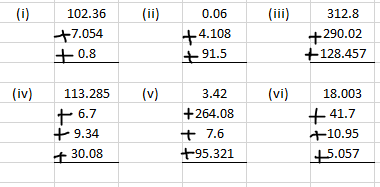Solution:

(i) We know that

102.360 + 7.054 + 0.800 = 110.214

(ii) We know that

0.060 + 4.108 + 91.500 = 95.668

(iii) We know that

312.800 + 290.020 + 128.457 = 731.277

(iv) We know that

113.285 + 6.700 + 9.340 + 30.080 = 159.405

(v) We know that

3.42 + 264.08 + 7.6 + 95.321 = 370.421

(vi) We know that

18.003 + 41.700 + 10.950 + 5.057 = 75.710

(i) 41.8, 39.24, 5.01 and 62.6

(ii) 4.702, 4.2, 6.02 and 1.27

(iii) 18.03, 146.3, 0.829 and 5.324

Solution:

(i) 41.8, 39.24, 5.01 and 62.6

It can be written as

41.80 + 39.24 + 5.01 + 62.60 = 148.65

(ii) 4.702, 4.2, 6.02 and 1.27

It can be written as

4.702 + 4.200 + 6.020 + 1.270 = 16.192

(iii) 18.03, 146.3, 0.829 and 5.324

It can be written as

18.030 + 146.300 + 0.829 + 5.324 = 170.483

3. Find the sum in each of the following:

(i) 0.007 + 8.5 + 30.08

(ii) 280.69 + 25.2 + 38

(iii) 25.65 + 9.005 + 3.7

(iv) 27.076 + 0.55 + 0.004

Solution:

(i) 0.007 + 8.5 + 30.08

It can be written as

0.007 + 8.500 + 30.080 = 38.587

(ii) 280.69 + 25.2 + 38

It can be written as

280.69 + 25.20 + 38.00 = 343.89

(iii) 25.65 + 9.005 + 3.7

It can be written as

25.650 + 9.005 + 3.700 = 38.355

(iv) 27.076 + 0.55 + 0.004

It can be written as

27.076 + 0.550 + 0.004 = 27.630

4. Radhika’s mother gave her Rs 10.50 and her father gave her Rs 15.80, find the total amount given to Radhika by her parents.

Solution:

Amount given by Radhika’s mother = Rs 10.50

Amount given by Radhika’s father = Rs 15.80

So the total amount given by her parents = Rs 10.50 + Rs 15.80 = Rs 26.30

Hence, the total amount given by her parents is Rs 26.30.

5. Rahul bought 4 kg 90 g apples, 2 kg 60 g of grapes and 5 kg 300 g of mangoes. Find the weight of the fruits he bought in all.

Solution:

Weight of apples bought by Rahul = 4 kg 90 g = 4.090 kg

Weight of grapes bought by Rahul = 2 kg 60 g = 2.060 kg

Weight of mangoes bought by Rahul = 5 kg 300 g = 5.300 kg

So the weight of all the fruits = 4.090 + 2.060 + 5.300 = 11.450 kg

Hence, the weight of the fruits bought by Rahul is 11.450 kg.

6. Nasreen bought 3 m 20 cm cloth for her shirt and 2 m 5 cm cloth for skirt. Find the total cloth bought by her.

Solution:

Cloth bought by Nasreen for shirt = 3 m 20 cm = 3.20 m

Cloth bought by Nasreen for skirt = 2 m 50 cm = 2.05 m

So the total cloth bought by her = 3.20 + 2.05 = 5.25 m = 5 m 25 cm

Hence, the total cloth bought by her is 5 m 25 cm.

7. Sunita travels 15 km 268 m by bus, 7 km 7 m by car and 500 m by foot in order to reach her school. How far is her school from her residence?

Solution:

Distance travelled by Sunita by bus = 15 km 268 m = 15.268 km

Distance travelled by Sunita by car = 7 km 7 m = 7.007 km

Distance travelled by Sunita by foot = 500 m = 0.500 km

So the distance from residence to school = 15.268 + 7.007 + 0.500 = 22.775 km

Hence, the distance from her residence to school is 22.775 km.

### Exercise 7.9 page: 7.31

1. Subtract: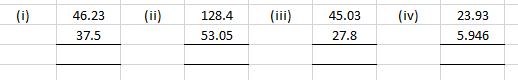Solution:

(i) We know that

46.23 – 37.5 = 8.73

(ii) We know that

128.4 – 53.05 = 75.35

(iii) We know that

45.03 – 27.8 = 17.23

(iv) We know that

23.93 – 5.946 = 17.984

2. Find the value of:

(i) 9.756 – 6.28

(ii) 21.05 – 15.27

(iii) 18.5 – 6.79

(iv) 48.1 – 0.37

(v) 108.032 – 86.8

(vi) 91.001 – 72.9

(vii) 32.7 – 25.86

(viii) 100 – 26.32

Solution:

(i) 9.756 – 6.28

We know that

9.756 – 6.280 = 3.476

(ii) 21.05 – 15.27

We know that

21.05 – 15.27 = 5.78

(iii) 18.5 – 6.79

We know that

18.50 – 6.79 = 11.71

(iv) 48.1 – 0.37

We know that

48.10 – 0.37 = 47.73

(v) 108.032 – 86.8

We know that

108.032 – 86.800 = 21.232

(vi) 91.001 – 72.9

We know that

91.001 – 72.900 = 18.101

(vii) 32.7 – 25.86

We know that

32.70 – 25.86 = 6.84

(viii) 100 – 26.32

We know that

100 – 26.32 = 73.68

3. The sum of two numbers is 100. If one of them is 78.01, find the other.

Solution:

One of the number = 78.01

Sum of two numbers = 100

Consider x as the other number

It can be written as

78.01 + x = 100

On further calculation

x = 100 – 78.01

By subtraction

x = 21.99

Hence, the other number is 21.99.

4. Waheeda’s school is at a distance of 5 km 350 m from her house. She travels 1 km 70 m on foot and the rest she travels by bus. How much distance does she travel by bus?

Solution:

Distance of school from house = 5 km 350 m = 5.350 km

Distance travelled on foot = 1 km 70 m = 1.070 km

Consider x km as the distance travelled by bus

It can be written as

1.070 + x = 5.350

On further calculation

x = 5.350 – 1.070

So we get

x = 4.280 km

Hence, the distance travelled by bus is 4.280 km.

5. Raju bought a book for Rs 35.65. He gave Rs 50 to the shopkeeper. How much money did he get back from the shopkeeper?

Solution:

Cost of book = Rs 35.65

Amount given = Rs 50

So the balance returned = 50 – 35.65 = Rs 14.35

Hence, the balance returned by the shopkeeper is Rs 14.35.

6. Ruby bought a watermelon weighing 5 kg 200 g. Out of this she gave 2 kg 750 g to her neighbour. What is the weight of the watermelon left with Ruby?

Solution:

Weight of watermelon bought by Ruby = 5 kg 200 g = 5.200 kg

Weight of watermelon Ruby gave to neighbour = 2 kg 750 g = 2.750 kg

So the weight of watermelon left with Ruby = Weight of watermelon bought by Ruby – Weight of watermelon Ruby gave to neighbour

We get

Weight of watermelon left with Ruby = 5.200 – 2.750 = 2.450 kg

Hence, the weight of watermelon left with Ruby is 2.450 kg.

7. Victor drove 89.050 km on Saturday and 73.9 km on Sunday. How many kilometres more did he drive on Sunday?

Solution:

Distance travelled by Victor on Saturday = 89.050 km

Distance travelled by Victor on Sunday = 73.9 km

So the distance travelled more by Victor on Saturday = 89.050 – 73.9 = 15.15 km

Hence, Victor drove 15.15 km more on Saturday.

8. Raju bought a book for Rs 35.65. He gave Rs 50 to the shopkeeper. How much money did he get back from the shopkeeper?

Solution:

Cost of the book = Rs 35.65

Amount given = Rs 50

So the balance retuned = 50 – 35.65 = Rs 14.35

Hence, the shopkeeper returned back Rs 14.35.

9. Gopal travelled 125.5 km by bus, 14.25 km by pony and the rest of distance to Kedarnath on foot. If he covered a total distance of 15 km, how much did he travel on foot?

Solution:

Distance travelled by Gopal by bus = 125.5 km

Distance travelled by Gopal by pony = 14.25km

Consider x km as the distance travelled on foot

We know that

Total distance = Distance travelled by Gopal by bus + Distance travelled by Gopal by pony + Distance travelled by Gopal on foot

By substituting the values

150 = 125.5 + 14.25 + x

On further calculation

x = 150 – 125.5 – 14.25

We get

x = 10.25 km

Hence, the distance travelled by Gopal on foot is 10.25 km.

10. Tina had 20 m 5 cm long cloth. She cuts 4 m 50 cm length of cloth from this for making a curtain. How much cloth is left with her?

Solution:

Length of cloth Tina had = 20 m 5 cm = 20.05 m

Length of cloth cut to make curtain = 4m 50 cm = 4.50 m

So the length of cloth left with her = Length of cloth Tina had – Length of cloth cut to make curtain

By substituting values

The length of cloth left with her = 20.05 – 4.50 = 15.55 m

Hence, the length of cloth left with Tina is 15.55 m.

11. Vineeta bought a book for Rs 18.90, a pen for Rs 8.50 and some papers for Rs 5.05. She gave fifty rupee to the shopkeeper. How much balance did she get back?

Solution:

Cost of book = Rs 18.90

Cost of pen = Rs 8.50

Cost of papers = Rs 5.05

So the total cost = 18.90 + 8.50 + 5.05 = Rs 32.45

Amount given to the shopkeeper = Rs 50

So the balance returned back = 50 – 32.45 = Rs 17.55

Hence, the shopkeeper returned back Rs 17.55.

12. Tanuj walked 8.62 km on Monday, 7.05 km on Tuesday and some distance on Wednesday. If he walked 21.01 km in three days, how much distance did he walk on Wednesday?

Solution:

Distance walked by Tanuj on Monday = 8.62 km

Distance walked by Tanuj on Tuesday = 7.05 km

Consider x km as the distance walked by Tanuj on Wednesday

It can be written as

Total distance = Distance walked by Tanuj on Monday + Distance walked by Tanuj on Tuesday + Distance walked by Tanuj on Wednesday

By substituting the values

21.01 = 8.62 + 7.05 + x

On further calculation

x = 21.01 – 8.62 – 7.05

So we get

x = 5.34 km

Hence, the distance walked by Tanuj on Wednesday is 5.34 km.

### Objective Type Questions page: 7.32

Mark the correct alternative in each of the following:

1. 3/10 is equal to
(a) 3.1
(b) 1.3
(c) 0.3
(d) 0.03

Solution:

The option (c) is correct answer.

We know that 3/10 = 0.3

Here the denominator is 10, so we have to mark the decimal where 3 is in the tenth place.

2. 7/100 is equal to
(a) 7.1
(b) 7.01
(c) 0.7
(d) 0.07

Solution:

The option (d) is correct answer.

We know that 7/100 = 0.07

Here the denominator is 100, so we have to mark the decimal where 7 is in the hundredth place.

3. 4/1000 is equal to
(a) 0.004
(b) 0.04
(c) 0.4
(d) 4.001

Solution:

The option (a) is correct answer.

Here the denominator is 1000, so we have to mark the decimal where 4 is in the thousandth place.

4. The value of 37/10000 is
(a) 0.0370
(b) 0.0037
(c) 0.00037
(d) 0.000037

Solution:

The option (b) is correct answer.

Here the denominator is 10000, so we have to mark the decimal where 3 is in the thousandth place and 7 is in the ten-thousandth place.

5. The place value of 5 in 0.04532 is
(a) 5
(b) 5/100
(c) 5/1000
(d) 5/10000

Solution:

The option (c) is correct answer.

We know that 5 is in the thousandth place.

So we get 0.04532 = 4/100 + 5/1000 + 3/10000 + 2/100000

6. The value of 231/1000 is
(a) 0.231
(b) 2.31
(c) 23.1
(d) 0.0231

Solution:

The option (a) is correct answer.

It can be written as

231/1000 = (200+30+1)/1000 = 200/1000 + 30/1000 + 1/1000 = 2/10 + 3/100 + 1/1000

Here we have 2 tenths, 3 hundredths and 1 thousandth.

Hence, the value of 231/1000 is 0.231.

7. The value of 3 5/1000 is
(a) 3.5
(b) 3.05
(c) 3.005
(d) 3.0005

Solution:

The option (c) is correct answer.

It can be written as

3 5/1000 = 3 + 5/1000 = 3 + 0.005 = 3.005

8. The value of 3/25 is
(a) 1.2
(b) 0.12
(c) 0.012
(d) None of these

Solution:

The option (b) is correct answer.

It can be written as

3/25 = (3×4)/ (25×4) = 12/100 = 0.12

9. The value of 2 1/25 is
(a) 2.4
(b) 2.25
(c) 2.04
(d) 2.40

Solution:

The option (c) is correct answer.

It can be written as

2 1/25 = 2 + 1/25 = 2 + (1×4)/ (25×4) = 2 + 4/100 = 2 + 0.04 = 2.04

10. 4 7/8 is equal to
(a) 4.78
(b) 4.87
(c) 4.875
(d) None of these

Solution:

The option (c) is correct answer.

It can be written as

4 7/8 = 4 + 7/8 = 4 + (7×125)/ (8×125)

On further calculation

4 7/8 = 4 + 875/1000 = 4 + 0.875 = 4.875

11. 2+3/10+5/100 is equal to
(a) 2.305
(b) 2.3
(c) 2.35
(d) 0.235

Solution:

The option (c) is correct answer.

We know that 3/10 = 0.3 having denominator as 10, so we need to mark the decimal where 3 is in the tenth place

5/100= 0.05 having denominator as 100, so we need to mark the decimal where 5 is in the hundredth place

It can be written as,

2 + 3/10 + 5/100 = 2+ 0.3 + 0.05 = 2.35

12. 3/100+5/10000 is equal to
(a) 0.35
(b) 0.305
(c) 0.0305
(d) 0.3005

Solution:

The option (c) is correct answer.

We know that 3/100 = 0.03 having denominator 100, so we mark the decimal where 3 is in the hundredth place

5/10000 = 0.0005 having denominator 10000, so we mark the decimal where 5 is in the ten thousandth place

It can be written as,

3/100 + 5/10000 = 0.03 + 0.0005 = 0.0305

13. 1 cm is equal is
(a) 0.1 m
(b) 0.01 m
(c) 0.10 m
(d) 0.001 m

Solution:

The option (b) is correct answer.

100 cm = 1 m

So we get,

1 cm = 1/100 m = 0.01 m

14. 1 m is equal to
(a) 0.1 km
(b) 0.01 km
(c) 0.001 km
(d) 0.0001 km

Solution:

The option (c) is correct answer.

1000 m = 1 km

So we get,

1 m = 1/1000 m = 0.001 km

15. 2 kg 5 gm is equal to
(a) 2.5 kg
(b) 2.05 kg
(c) 2.005 kg
(d) 2.6 kg

Solution:

The option (c) is correct answer.

1000 g = 1 kg

So we get 1 g = 1/1000 kg = 0.001 kg

The same way 5 g = 5/1000 kg = 0.005 kg

Hence, 2 kg 5 gm =2 kg + 0.005 kg = 2.005 kg

16. 15 litres and 15 ml is equal to
(a) 15.15 litres
(b) 15.150 litres
(c) 15.0015 litres
(d) 15.015 litres

Solution:

The option (d) is correct answer.

1000 ml = 1 litre

So we get 1 ml = 1/1000 = 0.001 litre

The same way 15 ml = 15/1000 = 0.015 litre

Hence, 15 litre and 15 ml = 15 litre + 0.015 litre = 15.015 litres

17. Which of the following are like decimals?
(a) 5.5, 5.05, 5.005, 5.50
(b) 5.5, 0.55, 5.55, 5.555
(c) 5.5, 6.6, 7.7, 8.8
(d) 0.5, 0.56, 0.567, 0.5678

Solution:

The option (c) is correct answer.

5.5. 6.6, 7.7, 8.8 are like decimals having same number of decimals.

18. The value of 0.5 + 0.005 + 5.05 is
(a) 5.55
(b) 5.555
(c) 5.055
(d) 5.550

Solution:

The option (b) is correct answer.

0.5 + 0.005 + 5.05 = 5.555

19. 0.35 − 0.035 is equal to
(a) 0.3
(b) 0.349
(c) 0.315
(d) 0.353

Solution:

The option (c) is correct answer.

0.35 – 0.035 = 0.315

20. 2.5 + 3.05 − 4.005 is equal to
(a) 1.545
(b) 1.455
(c) 1.554
(d) 0.545

Solution:

The option (a) is correct answer.

2.5 + 3.05 − 4.005 = 5.55 − 4.005 = 1.545

21. Which is greater among 2.3, 2.03, 2.33, 2.05?
(a) 2.3
(b) 2.03
(c) 2.33
(d) 2.05

Solution:

The option (c) is correct answer.

We know that the whole parts of all the above numbers are equal.

So by comparing the tenth parts, two of the decimals have a tenth part 0 and two have a tenth part 3.

Now by leaving the decimals which have a tenth part 0, 2.3 and 2.33.

By comparing them, 2.33 is greater than 2.3, where, 2.3 has no hundredth part, while 2.33, the hundredth part is 3.

### RD Sharma Solutions for Class 6 Maths Chapter 7: Decimals

Chapter 7, Decimals, has 9 exercises which explains the methods of solving problems along with shortcut tips which are essential. RD Sharma Solutions Chapter 7 helps us understand some of the major concepts which are mentioned below:

• Introduction
• Decimals as an extension of place value table
• Decimals as Fractions
• Expressing Fractions as Decimals
• Expressing Decimals as Fractions
• Comparing Decimals
• Some uses of Decimal Notation
• Equivalent Decimals
• Like and Unlike Decimals
• Addition of Numbers with Decimals
• Subtraction of Numbers with Decimals

### Chapter Brief of RD Sharma Solutions Class 6 Maths Chapter 7 – Decimals

The students can solve problems of RD Sharma textbook for Class 6 using the solutions as a reference material. This mainly improves confidence among students in solving tricky and lengthy problems easily. The PDF is easily accessible by the students and can be used as a reference material which speeds up exam preparation.

We use Decimals in daily lives, when we deal with money, length and weight of materials etc. It provides accurate value of required things. Here, by downloading PDF of solutions, the students can understand the concepts which are covered in this Chapter and methods of solving problems.